# woo.dem¶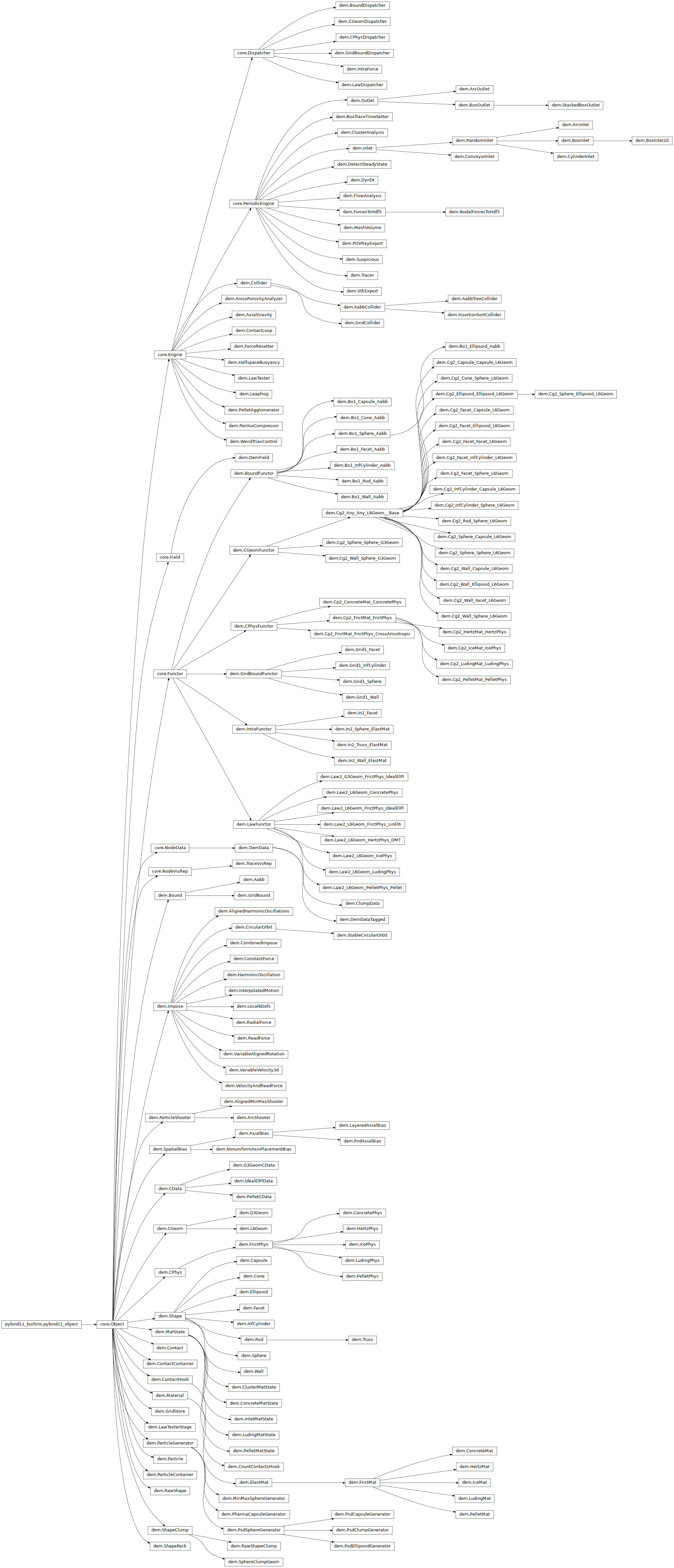## Stress/strain control¶

TODO

### PeriIsoCompressor¶

class woo.dem.PeriIsoCompressor(*args, **kwargs)

Compress/decompress cloud of spheres by controlling periodic cell size until it reaches prescribed average stress, then moving to next stress value in given stress series.

1. __init__(self: woo.dem.PeriIsoCompressor) -> None

2. __init__(self: woo.dem.PeriIsoCompressor, *args, **kwargs) -> None

stresses(= [])

Stresses that should be reached, one after another

[type: vector<Real>]

charLen(= -1.0)

Characteristic length, should be something like mean particle diameter (default -1=invalid value))

[type: Real]

maxSpan(= -1.0)

Maximum body span in terms of bbox, to prevent periodic cell getting too small.

maxUnbalanced(= 0.0001)

if actual unbalanced force is smaller than this number, the packing is considered stable,

[type: Real]

globalUpdateInt(= 20)

how often to recompute average stress, stiffness and unbalanced force

[type: int]

state(= 0)

Where are we at in the stress series

[type: size_t]

doneHook(= '')

Python command to be run when reaching the last specified stress

[type: string]

keepProportions(= True)

Exactly keep proportions of the cell (stress is controlled based on average, not its components

[type: bool]

currUnbalanced(= nan)

Current unbalanced force (updated internally)

avgStiffness(= nan)

Value of average stiffness (updated internally)

sigma(= Vector3(nan, nan, nan))

Current value of average stress (update internally)

### WeirdTriaxControl¶

class woo.dem.WeirdTriaxControl(*args, **kwargs)

Engine for independently controlling stress or strain in periodic simulations.

strainStress contains absolute values for the controlled quantity, and stressMask determines meaning of those values (0 for strain, 1 for stress): e.g. ( 1<<0 | 1<<2 ) = 1 | 4 = 5 means that strainStress and strainStress are stress values, and strainStress is strain.

See scripts/test/periodic-triax.py for a simple example.

1. __init__(self: woo.dem.WeirdTriaxControl) -> None

2. __init__(self: woo.dem.WeirdTriaxControl, *args, **kwargs) -> None

goal(= Vector3(0, 0, 0))

Desired stress or strain values (depending on stressMask), strains defined as strain(i)=log(Fii).

Warning

Strains are relative to the woo.core.Scene.cell.refSize (reference cell size), not the current one (e.g. at the moment when the new strain value is set).

[type: Vector3r]

stressMask(= 0)

mask determining strain/stress (0/1) meaning for goal components

[type: int]

maxStrainRate(= Vector3(1, 1, 1))

Maximum strain rate of the periodic cell.

[type: Vector3r]

maxUnbalanced(= 0.0001)

maximum unbalanced force.

[type: Real]

absStressTol(= 1000.0)

Absolute stress tolerance

[type: Real]

relStressTol(= 3e-05)

Relative stress tolerance; if negative, it is relative to the largest stress value along all axes, where strain is prescribed.

[type: Real]

maxStrainedStress(= nan)

Current abs-maximum stress in strain-controlled directions; useda as reference when relStressTol is negative.

growDamping(= 0.25)

Damping of cell resizing (0=perfect control, 1=no control at all).

[type: Real]

relVol(= 1.0)

For stress computation, use volume of the periodic cell multiplied by this constant.

[type: Real]

globUpdate(= 5)

How often to recompute average stress, stiffness and unbalaced force.

[type: int]

doneHook(= '')

python command to be run when the desired state is reached

[type: string]

stress(= Matrix3(0, 0, 0, 0, 0, 0, 0, 0, 0))

Stress tensor

[type: Matrix3r]

strain(= Vector3(0, 0, 0))

cell strain, updated automatically

[type: Vector3r]

strainRate(= Vector3(0, 0, 0))

cell strain rate, updated automatically

[type: Vector3r]

currUnbalanced(= nan)

current unbalanced force (updated every globUpdate)

[type: Real]

prevGrow(= Vector3(0, 0, 0))

previous cell grow

[type: Vector3r]

mass(= nan)

mass of the cell (user set); if not set, it will be computed as sum of masses of all particles.

[type: Real]

externalWork(= 0.0)

Work input from boundary controller.

[type: Real]

gradVWorkIx(= -1)

Index for work done by velocity gradient, if tracking energy

[type: int, not saved, not accessible from python]

leapfrogChecked(= False)

Whether we already checked that we come before Leapfrog (otherwise setting nextGradV will have no effect.

[type: bool, not accessible from python]

## Contacts¶

TODO

### Contact¶

class woo.dem.Contact(*args, **kwargs)

Contact in DEM

1. __init__(self: woo.dem.Contact) -> None

2. __init__(self: woo.dem.Contact, *args, **kwargs) -> None

geom(= None)

Contact geometry

[type: shared_ptr<CGeom>, read-only in python]

phys(= None)

Physical properties of contact

[type: shared_ptr<CPhys>, read-only in python]

data(= None)

Optional data stored by the functor for its own use

[type: shared_ptr<CData>, read-only in python]

pA

First particle of the contact

[type: weak_ptr<Particle>, read-only in python]

pB

Second particle of the contact

[type: weak_ptr<Particle>, read-only in python]

cellDist(= Vector3i(0, 0, 0))

Distace in the number of periodic cells by which pB must be shifted to get to the right relative position.

color(= 0.0)

(Normalized) color value for this contact

[type: Real]

stepCreated(= -1)

Step in which this contact was created by the collider, or step in which it was made real (if geom and phys exist). This number is NOT reset by Contact::reset(). If negative, it means the collider does not want to keep this contact around anymore (this happens if the contact is real but there is no overlap anymore).

minDist00Sq(= -1.0)

Minimum distance between nodes of both shapes so that the contact can exist. Set in ContactLoop by geometry functor once, and is used to check for possible contact without having to call the functor. If negative, not used. Currently, only Sphere-Sphere contacts use this information.

stepLastSeen(= -1)

linIx(= 0)

Position in the linear view (ContactContainer)

[type: size_t, not shown in the UI, read-only in python]

dPos(self: woo.dem.Contact)_wooEigen11.Vector3

Return position difference vector pB-pA, taking Contact.cellDist in account properly. Both particles must be uninodal, exception is raised otherwise.

dist(self: woo.dem.Contact)float

Shorthand for dPos.norm().

forceSign(*args, **kwargs)

1. forceSign(self: woo.dem.Contact, p: woo.dem.Particle) -> int

Return sign of CPhys.force as it appies on the particle passed, i.e. +1 if p==C.pA and -1 if p==C.pB. Raise an exception if p is neither pA or pB.

1. forceSign(self: woo.dem.Contact, id: int) -> int

Return sign of CPhys.force as it appies on the particle with id id, i.e. id==C.id1 and -1 if id==id2. Raise an exception if id is neither id1 or id2.

property id1

Particle.id of the first contacting particle.

property id2

Particle.id of the second contacting particle.

property ids

IDs of both contacting particles as 2-tuple.

isFresh(self: woo.dem.Contact, scene: woo.core.Scene)bool

Say whether this contact has just been created. Equivalent to C.stepCreated==scene.step.

property real

Whether the contact is real (has geom and phys); unreal contacts are created by broadband collisions detection and have no physical significance.

resetPhys(self: woo.dem.Contact)None

Set phys to None (to force its re-evaluation)

### ContactLoop¶

class woo.dem.ContactLoop(*args, **kwargs)

Loop over all contacts, possible in a parallel manner.

Special constructor

Constructs from 3 lists of Cg2, Cp2, Law functors respectively; they will be passed to interal dispatchers.

1. __init__(self: woo.dem.ContactLoop) -> None

2. __init__(self: woo.dem.ContactLoop, *args, **kwargs) -> None

geoDisp(= <CGeomDispatcher @ 0x1601b50>)

CGeomDispatcher object that is used for dispatch.

[type: shared_ptr<CGeomDispatcher>, read-only in python]

phyDisp(= <CPhysDispatcher @ 0x15f3ba0>)

CPhysDispatcher object used for dispatch.

[type: shared_ptr<CPhysDispatcher>, read-only in python]

lawDisp(= <LawDispatcher @ 0x1601a70>)

LawDispatcher object used for dispatch.

[type: shared_ptr<LawDispatcher>, read-only in python]

hook(= None)

ContactHook objects, empty by default.

[type: shared_ptr<ContactHook>]

alreadyWarnedNoCollider(= False)

Keep track of whether the user was already warned about missing collider.

[type: bool, not shown in the UI]

evalStress(= False)

Evaluate stress tensor, in periodic simluations; if energy tracking is enabled, increments gradV energy.

[type: bool]

applyForces(= True)

Apply forces directly; this avoids IntraForce engine, but will silently skip multinodal particles.

[type: bool]

updatePhys(= 0)

Call CPhysFunctor even for contacts which already have Contact.phys (to reflect changes in particle’s material, for example). ‘once’ will update only once and then set this back to ‘never’.

[type: int, named enum, possible values are: ‘never’ (0), ‘always’ (1), ‘once’ (2)]

dist00(= True)

Whether to apply the Contact.minDist00Sq optimization (for mesuring the speedup only)

[type: bool]

stress(= Matrix3(0, 0, 0, 0, 0, 0, 0, 0, 0))

Stress value, used to compute gradV energy if trackWork is True.

reorderEvery(= 1000)

Reorder contacts so that real ones are at the beginning in the linear sequence, making the OpenMP loop traversal (hopefully) less unbalanced.

[type: int]

prevVol(= nan)

Previous value of cell volume

[type: Real, not accessible from python]

prevStress(= Matrix3(0, 0, 0, 0, 0, 0, 0, 0, 0))

Previous value of stress, used to compute mid-step stress

[type: Matrix3r]

gradVIx(= -1)

Cache energy index for gradV work

[type: int, not saved, not accessible from python]

### ContactHook¶class woo.dem.ContactHook(*args, **kwargs)

Functor called from ContactLoop for some specific events on contacts; currently, these events are contact creation and contact deletion. This base class does nothing.

1. __init__(self: woo.dem.ContactHook) -> None

2. __init__(self: woo.dem.ContactHook, *args, **kwargs) -> None

mask(= 0)

Mask which must be matched by both particles in the contact.

[type: int]

#### CountContactsHook¶

class woo.dem.CountContactsHook(*args, **kwargs)

Functor counting contacts created and deleted in ContactLoop (contacts being deleted e.g. as a result of particle deletion are not counted), restricted by mask.

1. __init__(self: woo.dem.CountContactsHook) -> None

2. __init__(self: woo.dem.CountContactsHook, *args, **kwargs) -> None

nNew

Total number of new (real) contacts.

[type: OpenMPAccumulator<int>]

nDel

Total number of deleted contacts.

[type: OpenMPAccumulator<int>]

### Geometry¶

TODO

#### CGeom¶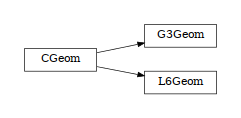class woo.dem.CGeom(*args, **kwargs)

Geometrical configuration of contact

1. __init__(self: woo.dem.CGeom) -> None

2. __init__(self: woo.dem.CGeom, *args, **kwargs) -> None

node(= <Node @ 0x15e9870, at (0, 0, 0)>)

Local coordinates definition.

[type: shared_ptr<Node>]

dispHierarchy(self: woo.dem.CGeom, names: bool = True)list

Return list of dispatch classes (from down upwards), starting with the class instance itself, top-level indexable at last. If names is true (default), return class names rather than numerical indices.

property dispIndex

Return class index of this instance.

#### L6Geom¶

class woo.dem.L6Geom(*args, **kwargs)

Geometry of particles in contact, defining relative velocities.

1. __init__(self: woo.dem.L6Geom) -> None

2. __init__(self: woo.dem.L6Geom, *args, **kwargs) -> None

vel(= Vector3(0, 0, 0))

Relative displacement rate in local coordinates, defined by CGeom.node

[type: Vector3r, unit: m/s]

angVel(= Vector3(0, 0, 0))

Relative rotation rate in local coordinates

uN(= nan)

Normal displacement, distace of separation of particles (mathematically equal to integral of vel, but given here for numerically more stable results, as this component can be usually computed directly).

[type: Real]

lens(= Vector2(0, 0))

Hint for Cp2 functor on how to distribute material stiffnesses according to lengths on both sides of the contact; their sum should be equal to the initial contact length.

[type: Vector2r, unit: m]

contA(= nan)

(Fictious) contact area, used by Cp2 functor to compute stiffness.

[type: Real, unit: m²]

trsf(= Matrix3(1, 0, 0, 0, 1, 0, 0, 0, 1))

Transformation (rotation) from global to local coordinates; only used internally, and is synchronized with woo.core.Node.ori automatically. If the algorithm works with pure quaternions at some point (it is not stable now), can be removed safely.

[type: Matrix3r]

#### G3Geom¶

class woo.dem.G3Geom(*args, **kwargs)

Geometry of particles in contact, defining relative velocities.

1. __init__(self: woo.dem.G3Geom) -> None

2. __init__(self: woo.dem.G3Geom, *args, **kwargs) -> None

uN(= nan)

Normal displacement, distace of separation of particles (mathematically equal to integral of vel, but given here for numerically more stable results, as this component can be usually computed directly).

[type: Real]

dShear(= Vector3(0, 0, 0))

Shear displacement delta during last step.

[type: Vector3r]

twistAxis(= Vector3(nan, nan, nan))

Axis of twisting rotation

orthonormalAxis(= Vector3(nan, nan, nan))

Axis normal to twisting axis

normal(= Vector3(nan, nan, nan))

Contact normal in global coordinates; G3Geom doesn’t touch Contact.node.ori (which is identity), therefore orientation must be kep separately

#### CGeomFunctor¶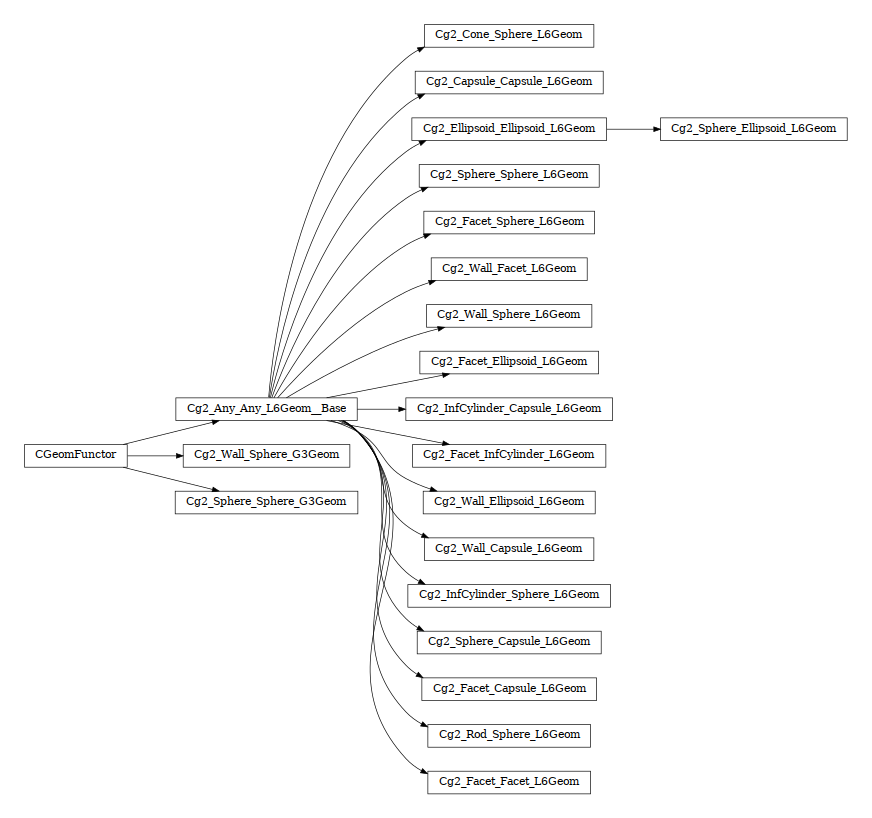class woo.dem.CGeomFunctor(*args, **kwargs)

Functor for creating/updating Contact.geom objects.

1. __init__(self: woo.dem.CGeomFunctor) -> None

2. __init__(self: woo.dem.CGeomFunctor, *args, **kwargs) -> None

##### Cg2_Sphere_Sphere_G3Geom¶
class woo.dem.Cg2_Sphere_Sphere_G3Geom(*args, **kwargs)

Incrementally compute G3Geom for contact of 2 spheres. Detailed documentation in py/_extraDocs.py

1. __init__(self: woo.dem.Cg2_Sphere_Sphere_G3Geom) -> None

2. __init__(self: woo.dem.Cg2_Sphere_Sphere_G3Geom, *args, **kwargs) -> None

noRatch(= True)

FIXME: document what it really does.

[type: bool]

useAlpha(= True)

Use alpha correction proposed by McNamara, see source code for details

[type: bool]

##### Cg2_Any_Any_L6Geom__Base¶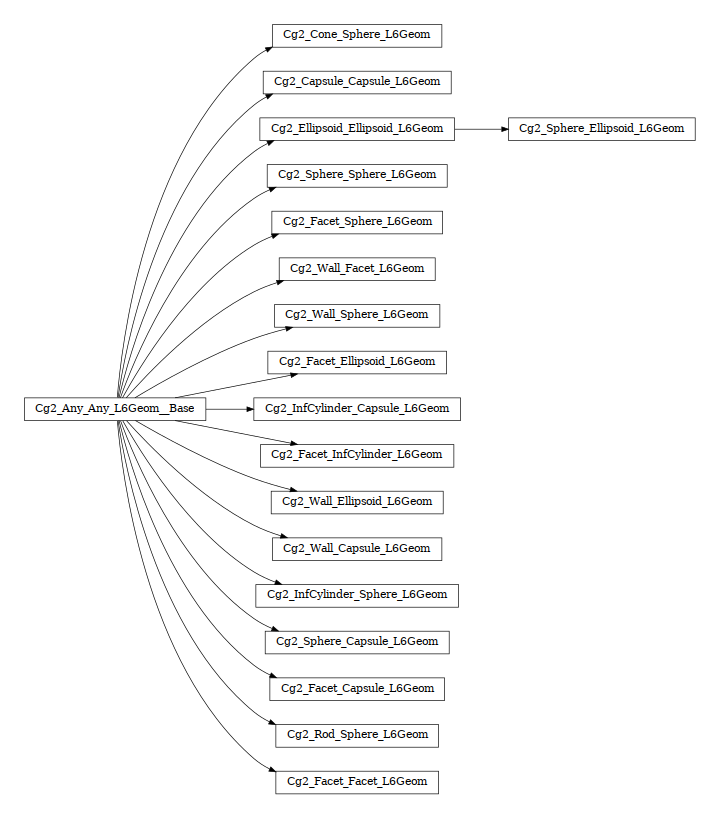class woo.dem.Cg2_Any_Any_L6Geom__Base(*args, **kwargs)

Common base for L6Geom-computing functors such as Cg2_Sphere_Sphere_L6Geom, holding common approximation flags.

1. __init__(self: woo.dem.Cg2_Any_Any_L6Geom__Base) -> None

2. __init__(self: woo.dem.Cg2_Any_Any_L6Geom__Base, *args, **kwargs) -> None

noRatch(= False)

FIXME: document what it really does.

[type: bool]

iniLensTouch(= True)

Set L6Geom.lens to touch distance (e.g. radii sum for spheres). If false, L6Geom.lens is set to the initial contact distance (which is less than the touch distance, with overlap subtracted); this implies path-dependent L6Geom.lens and unpredictable stiffness. Do not set to false unless you know what you are doing.

[type: bool]

trsfRenorm(= 100)

How often to renormalize trsf; if non-positive, never renormalized (simulation might be unstable)

[type: int]

approxMask(= 0)

Selectively enable geometrical approximations (bitmask); add the values for approximations to be enabled.

 1 use previous normal instead of mid-step normal for computing tangent velocity 2 do not re-normalize average (mid-step) normal, if used. 4 use previous rotation instead of mid-step rotation to transform velocities 8 use current branches instead of mid-step branches to evaluate incident velocity (used without noRatch)

By default, the mask is zero, wherefore none of these approximations is used.

[type: int, range: 0−15]

###### Cg2_Facet_Capsule_L6Geom¶
class woo.dem.Cg2_Facet_Capsule_L6Geom(*args, **kwargs)

Compute L6Geom for contact of Capsule and Facet.

1. __init__(self: woo.dem.Cg2_Facet_Capsule_L6Geom) -> None

2. __init__(self: woo.dem.Cg2_Facet_Capsule_L6Geom, *args, **kwargs) -> None

###### Cg2_Wall_Facet_L6Geom¶
class woo.dem.Cg2_Wall_Facet_L6Geom(*args, **kwargs)

Incrementally compute L6Geom for contact between Wall and Facet.

1. __init__(self: woo.dem.Cg2_Wall_Facet_L6Geom) -> None

2. __init__(self: woo.dem.Cg2_Wall_Facet_L6Geom, *args, **kwargs) -> None

###### Cg2_Facet_InfCylinder_L6Geom¶
class woo.dem.Cg2_Facet_InfCylinder_L6Geom(*args, **kwargs)

Incrementally compute L6Geom for contact between Facet and InfCylinder.

1. __init__(self: woo.dem.Cg2_Facet_InfCylinder_L6Geom) -> None

2. __init__(self: woo.dem.Cg2_Facet_InfCylinder_L6Geom, *args, **kwargs) -> None

###### Cg2_Wall_Capsule_L6Geom¶
class woo.dem.Cg2_Wall_Capsule_L6Geom(*args, **kwargs)

Compute L6Geom for contact of Capsule and Wall (axis-aligned plane).

1. __init__(self: woo.dem.Cg2_Wall_Capsule_L6Geom) -> None

2. __init__(self: woo.dem.Cg2_Wall_Capsule_L6Geom, *args, **kwargs) -> None

###### Cg2_Wall_Sphere_L6Geom¶
class woo.dem.Cg2_Wall_Sphere_L6Geom(*args, **kwargs)

Incrementally compute L6Geom for contact between Wall and Sphere. Uses attributes of Cg2_Sphere_Sphere_L6Geom.

1. __init__(self: woo.dem.Cg2_Wall_Sphere_L6Geom) -> None

2. __init__(self: woo.dem.Cg2_Wall_Sphere_L6Geom, *args, **kwargs) -> None

###### Cg2_Facet_Facet_L6Geom¶
class woo.dem.Cg2_Facet_Facet_L6Geom(*args, **kwargs)

Incrementally compute L6Geom for contact between two Facet shapes.

1. __init__(self: woo.dem.Cg2_Facet_Facet_L6Geom) -> None

2. __init__(self: woo.dem.Cg2_Facet_Facet_L6Geom, *args, **kwargs) -> None

###### Cg2_Ellipsoid_Ellipsoid_L6Geom¶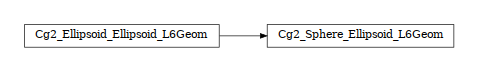class woo.dem.Cg2_Ellipsoid_Ellipsoid_L6Geom(*args, **kwargs)

Incrementally compute L6Geom for contact of 2 ellipsoids. Uses the Perram-Wertheim potential function ([PW85], , [DTS05]). See example scripts examples/ell0.py and examples/ell1.py.

1. __init__(self: woo.dem.Cg2_Ellipsoid_Ellipsoid_L6Geom) -> None

2. __init__(self: woo.dem.Cg2_Ellipsoid_Ellipsoid_L6Geom, *args, **kwargs) -> None

brent(= True)

Use Brent iteration for finding maximum of the Perram-Wertheim potential. If false, use Newton-Raphson (not yet implemented).

[type: bool]

brentBits(= 32)

Precision for the Brent method, as number of bits.

[type: int]

Cg2_Sphere_Ellipsoid_L6Geom
class woo.dem.Cg2_Sphere_Ellipsoid_L6Geom(*args, **kwargs)

Compute the geometry of Ellipsoid + Sphere collision. Uses the code from Cg2_Ellipsoid_Ellipsoid_L6Geom, representing the sphere as an ellipsoid with all semiaxes equal to the radius.

1. __init__(self: woo.dem.Cg2_Sphere_Ellipsoid_L6Geom) -> None

2. __init__(self: woo.dem.Cg2_Sphere_Ellipsoid_L6Geom, *args, **kwargs) -> None

###### Cg2_Sphere_Capsule_L6Geom¶
class woo.dem.Cg2_Sphere_Capsule_L6Geom(*args, **kwargs)

Compute L6Geom for contact of Capsule and Sphere.

1. __init__(self: woo.dem.Cg2_Sphere_Capsule_L6Geom) -> None

2. __init__(self: woo.dem.Cg2_Sphere_Capsule_L6Geom, *args, **kwargs) -> None

###### Cg2_Facet_Sphere_L6Geom¶
class woo.dem.Cg2_Facet_Sphere_L6Geom(*args, **kwargs)

Incrementally compute L6Geom for contact between Facet and Sphere.

1. __init__(self: woo.dem.Cg2_Facet_Sphere_L6Geom) -> None

2. __init__(self: woo.dem.Cg2_Facet_Sphere_L6Geom, *args, **kwargs) -> None

###### Cg2_Capsule_Capsule_L6Geom¶
class woo.dem.Cg2_Capsule_Capsule_L6Geom(*args, **kwargs)

Collision of two Capsule shapes.

1. __init__(self: woo.dem.Cg2_Capsule_Capsule_L6Geom) -> None

2. __init__(self: woo.dem.Cg2_Capsule_Capsule_L6Geom, *args, **kwargs) -> None

###### Cg2_Rod_Sphere_L6Geom¶
class woo.dem.Cg2_Rod_Sphere_L6Geom(*args, **kwargs)

Incrementally compute L6Geom for contact between Rod and Sphere. Uses attributes of Cg2_Sphere_Sphere_L6Geom.

1. __init__(self: woo.dem.Cg2_Rod_Sphere_L6Geom) -> None

2. __init__(self: woo.dem.Cg2_Rod_Sphere_L6Geom, *args, **kwargs) -> None

###### Cg2_Facet_Ellipsoid_L6Geom¶
class woo.dem.Cg2_Facet_Ellipsoid_L6Geom(*args, **kwargs)

Compute L6Geom for contact of ellipsoid and facet.

Warning

This class does not work (the result is correct only for face contact, otherwise bogus) and is by default not returned in woo.dem.DemField.minimalEngines. See this question for a brief discussion.

1. __init__(self: woo.dem.Cg2_Facet_Ellipsoid_L6Geom) -> None

2. __init__(self: woo.dem.Cg2_Facet_Ellipsoid_L6Geom, *args, **kwargs) -> None

###### Cg2_Cone_Sphere_L6Geom¶
class woo.dem.Cg2_Cone_Sphere_L6Geom(*args, **kwargs)

Incrementally compute L6Geom for contact between Cone and Sphere. Uses attributes of Cg2_Sphere_Sphere_L6Geom.

1. __init__(self: woo.dem.Cg2_Cone_Sphere_L6Geom) -> None

2. __init__(self: woo.dem.Cg2_Cone_Sphere_L6Geom, *args, **kwargs) -> None

###### Cg2_InfCylinder_Sphere_L6Geom¶
class woo.dem.Cg2_InfCylinder_Sphere_L6Geom(*args, **kwargs)

Incrementally compute L6Geom for contact between InfCylinder and Sphere. Uses attributes of Cg2_Sphere_Sphere_L6Geom.

1. __init__(self: woo.dem.Cg2_InfCylinder_Sphere_L6Geom) -> None

2. __init__(self: woo.dem.Cg2_InfCylinder_Sphere_L6Geom, *args, **kwargs) -> None

###### Cg2_InfCylinder_Capsule_L6Geom¶
class woo.dem.Cg2_InfCylinder_Capsule_L6Geom(*args, **kwargs)

Compute L6Geom for contact of Capsule and InfCylinder.

1. __init__(self: woo.dem.Cg2_InfCylinder_Capsule_L6Geom) -> None

2. __init__(self: woo.dem.Cg2_InfCylinder_Capsule_L6Geom, *args, **kwargs) -> None

###### Cg2_Sphere_Sphere_L6Geom¶
class woo.dem.Cg2_Sphere_Sphere_L6Geom(*args, **kwargs)

Functor for computing incrementally configuration of 2 Spheres stored in L3Geom; the configuration is positioned in global space by local origin $$\vec{c}$$ (contact point) and rotation matrix $$\mat{T}$$ (orthonormal transformation matrix), and its degrees of freedom are local displacement $$\vec{u}$$ (in one normal and two shear directions); with Ig2_Sphere_Sphere_L6Geom and L6Geom, there is additionally $$\vec{\phi}$$. The first row of $$\mat{T}$$, i.e. local $$x$$-axis, is the contact normal noted $$\vec{n}$$ for brevity. Additionally, quasi-constant values of $$\vec{u}_0$$ (and $$\vec{\phi}_0$$) are stored as shifted origins of $$\vec{u}$$ (and $$\vec{\phi}$$); therefore, current value of displacement is always $$\curr{\vec{u}}-\vec{u}_0$$.

Suppose two spheres with radii $$r_i$$, positions $$\vec{x}_i$$, velocities $$\vec{v}_i$$, angular velocities $$\vec{\omega}_i$$.

When there is not yet contact, it will be created if $$u_N=|\curr{\vec{x}}_2-\curr{\vec{x}}_1|-|f_d|(r_1+r2)<0$$, where $$f_d$$ is distFactor (sometimes also called “interaction radius”). If $$f_d>0$$, then $$\vec{u}_{0x}$$ will be initalized to $$u_N$$, otherwise to 0. In another words, contact will be created if spheres enlarged by $$|f_d|$$ touch, and the “equilibrium distance” (where $$\vec{u}_x-\vec{u}-{0x}$$ is zero) will be set to the current distance if $$f_d$$ is positive, and to the geometrically-touching distance if negative.

Local axes (rows of $$\mat{T}$$) are initially defined as follows:

• local $$x$$-axis is $$\vec{n}=\vec{x}_l=\normalized{\vec{x}_2-\vec{x}_1}$$;

• local $$y$$-axis positioned arbitrarily, but in a deterministic manner: aligned with the $$xz$$ plane (if $$\vec{n}_y<\vec{n}_z$$) or $$xy$$ plane (otherwise);

• local $$z$$-axis $$\vec{z}_l=\vec{x}_l\times\vec{y}_l$$.

If there has already been contact between the two spheres, it is updated to keep track of rigid motion of the contact (one that does not change mutual configuration of spheres) and mutual configuration changes. Rigid motion transforms local coordinate system and can be decomposed in rigid translation (affecting $$\vec{c}$$), and rigid rotation (affecting $$\mat{T}$$), which can be split in rotation $$\vec{o}_r$$ perpendicular to the normal and rotation $$\vec{o}_t$$ (“twist”) parallel with the normal:

$\pprev{\vec{o}_r}=\prev{\vec{n}}\times\curr{\vec{n}}.$

Since velocities are known at previous midstep ($$t-\Dt/2$$), we consider mid-step normal

$\pprev{\vec{n}}=\frac{\prev{\vec{n}}+\curr{\vec{n}}}{2}.$

For the sake of numerical stability, $$\pprev{\vec{n}}$$ is re-normalized after being computed, unless prohibited by approxMask. If approxMask has the appropriate bit set, the mid-normal is not compute, and we simply use $$\pprev{\vec{n}}\approx\prev{\vec{n}}$$.

Rigid rotation parallel with the normal is

$\pprev{\vec{o}_t}=\pprev{\vec{n}}\left(\pprev{\vec{n}}\cdot\frac{\pprev{\vec{\omega}}_1+\pprev{\vec{\omega}}_2}{2}\right)\Dt.$

Branch vectors $$\vec{b}_1$$, $$\vec{b}_2$$ (connecting $$\curr{\vec{x}}_1$$, $$\curr{\vec{x}}_2$$ with $$\curr{\vec{c}}$$ are computed depending on noRatch (see here).

\begin{align*} \vec{b}_1&=\begin{cases} r_1 \curr{\vec{n}} & \mbox{with noRatch} \\ \curr{\vec{c}}-\curr{\vec{x}}_1 & \mbox{otherwise} \end{cases} \\ \vec{b}_2&=\begin{cases} -r_2\curr{\vec{n}} & \mbox{with noRatch} \\ \curr{\vec{c}}-\curr{\vec{x}}_2 & \mbox{otherwise} \end{cases} \\ \end{align*}

Relative velocity at $$\curr{\vec{c}}$$ can be computed as

$\pprev{\vec{v}_r}=(\pprev{\vec{\tilde{v}}_2}+\vec{\omega}_2\times\vec{b}_2)-(\vec{v}_1+\vec{\omega}_1\times\vec{b}_1)$

where $$\vec{\tilde{v}}_2$$ is $$\vec{v}_2$$ without mean-field velocity gradient in periodic boundary conditions (see Cell.homoDeform). In the numerial implementation, the normal part of incident velocity is removed (since it is computed directly) with $$\pprev{\vec{v}_{r2}}=\pprev{\vec{v}_r}-(\pprev{\vec{n}}\cdot\pprev{\vec{v}_r})\pprev{\vec{n}}$$.

Any vector $$\vec{a}$$ expressed in global coordinates transforms during one timestep as

$\curr{\vec{a}}=\prev{\vec{a}}+\pprev{\vec{v}_r}\Dt-\prev{\vec{a}}\times\pprev{\vec{o}_r}-\prev{\vec{a}}\times{\pprev{\vec{t}_r}}$

where the increments have the meaning of relative shear, rigid rotation normal to $$\vec{n}$$ and rigid rotation parallel with $$\vec{n}$$. Local coordinate system orientation, rotation matrix $$\mat{T}$$, is updated by rows, i.e.

$\begin{split}\curr{\mat{T}}=\begin{pmatrix} \curr{\vec{n}_x}, \curr{\vec{n}_y}, \curr{\vec{n}_z} \\ \prev{\mat{T}_{1,\bullet}}-\prev{\mat{T}_{1,\bullet}}\times\pprev{\vec{o}_r}-\prev{\mat{T}_{1,\bullet}}\times\pprev{\vec{o}_t} \\ \prev{\mat{T}_{2,\bullet}}-\prev{\mat{T}_{2,\bullet}}\times\pprev{\vec{o}_r}-\prev{\mat{T}_{,\bullet}}\times\pprev{\vec{o}_t} \end{pmatrix}\end{split}$

This matrix is re-normalized (unless prevented by approxMask) and mid-step transformation is computed using quaternion spherical interpolation as

$\pprev{\mat{T}}=\mathrm{Slerp}\,\left(\prev{\mat{T}};\curr{\mat{T}};t=1/2\right).$

Depending on approxMask, this computation can be avoided by approximating $$\pprev{\mat{T}}=\prev{\mat{T}}$$.

Finally, current displacement is evaluated as

$\curr{\vec{u}}=\prev{u}+\pprev{\mat{T}}\pprev{\vec{v}_r}\Dt.$

For the normal component, non-incremental evaluation is preferred, giving

$\curr{\vec{u}_x}=|\curr{\vec{x}_2}-\curr{\vec{x}_1}|-(r_1+r_2)$

If this functor is called for L6Geom, local rotation is updated as

$\curr{\vec{\phi}}=\prev{\vec{\phi}}+\pprev{\mat{T}}\Dt(\vec{\omega}_2-\vec{\omega}_1)$

1. __init__(self: woo.dem.Cg2_Sphere_Sphere_L6Geom) -> None

2. __init__(self: woo.dem.Cg2_Sphere_Sphere_L6Geom, *args, **kwargs) -> None

distFactor(= -1.0)

removed in API 10103, set DemField.distFactor instead.

[type: Real, not shown in the UI, not dumped, DEPRECATED, raises ValueError when accessed]

###### Cg2_Wall_Ellipsoid_L6Geom¶
class woo.dem.Cg2_Wall_Ellipsoid_L6Geom(*args, **kwargs)

Compute L6Geom for contact of ellipsoid and wall (axis-aligned plane).

1. __init__(self: woo.dem.Cg2_Wall_Ellipsoid_L6Geom) -> None

2. __init__(self: woo.dem.Cg2_Wall_Ellipsoid_L6Geom, *args, **kwargs) -> None

##### Cg2_Wall_Sphere_G3Geom¶
class woo.dem.Cg2_Wall_Sphere_G3Geom(*args, **kwargs)

Incrementally compute G3Geom for contact of 2 spheres. Detailed documentation in py/_extraDocs.py

1. __init__(self: woo.dem.Cg2_Wall_Sphere_G3Geom) -> None

2. __init__(self: woo.dem.Cg2_Wall_Sphere_G3Geom, *args, **kwargs) -> None

#### CGeomDispatcher¶

class woo.dem.CGeomDispatcher(*args, **kwargs)

Dispatcher calling functors based on received argument type(s).

1. __init__(self: woo.dem.CGeomDispatcher) -> None

2. __init__(self: woo.dem.CGeomDispatcher, *args, **kwargs) -> None

functors(= [])

Functors active in the dispatch mechanism [overridden below].

[type: vector<shared_ptr<CGeomFunctor>>]

dispFunctor(self: woo.dem.CGeomDispatcher, arg0: woo.dem.Shape, arg1: woo.dem.Shape)woo.dem.CGeomFunctor

Return functor that would be dispatched for given argument(s); None if no dispatch; ambiguous dispatch throws.

dispMatrix(self: woo.dem.CGeomDispatcher, names: bool = True)dict

Return dictionary with contents of the dispatch matrix.

### Physical properties¶

TODO

#### CPhys¶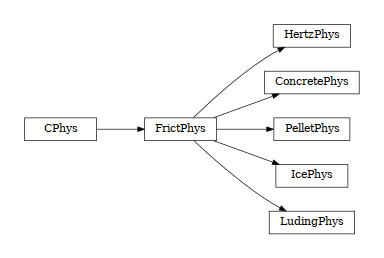class woo.dem.CPhys(*args, **kwargs)

Physical properties of contact.

1. __init__(self: woo.dem.CPhys) -> None

2. __init__(self: woo.dem.CPhys, *args, **kwargs) -> None

force(= Vector3(0, 0, 0))

Force applied on the first particle in the contact

[type: Vector3r, unit: N]

torque(= Vector3(0, 0, 0))

Torque applied on the first particle in the contact

[type: Vector3r, unit: N·m]

dispHierarchy(self: woo.dem.CPhys, names: bool = True)list

Return list of dispatch classes (from down upwards), starting with the class instance itself, top-level indexable at last. If names is true (default), return class names rather than numerical indices.

property dispIndex

Return class index of this instance.

#### FrictPhys¶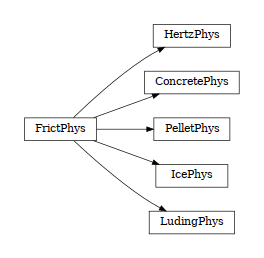class woo.dem.FrictPhys(*args, **kwargs)

Physical parameters of contact with sliding

1. __init__(self: woo.dem.FrictPhys) -> None

2. __init__(self: woo.dem.FrictPhys, *args, **kwargs) -> None

tanPhi(= nan)

Tangent of friction angle

[type: Real]

kn(= nan)

Normal stiffness

[type: Real]

kt(= nan)

Tangent stiffness

[type: Real]

##### PelletPhys¶
class woo.dem.PelletPhys(*args, **kwargs)

Physical properties of a contact of two PelletMat.

1. __init__(self: woo.dem.PelletPhys) -> None

2. __init__(self: woo.dem.PelletPhys, *args, **kwargs) -> None

normPlastCoeff(= nan)

Normal plasticity coefficient.

[type: Real]

ka(= nan)

[type: Real]

##### LudingPhys¶
class woo.dem.LudingPhys(*args, **kwargs)

Physical properties for luding-contact-model.

1. __init__(self: woo.dem.LudingPhys) -> None

2. __init__(self: woo.dem.LudingPhys, *args, **kwargs) -> None

kn1(= nan)

[type: Real]

kna(= nan)

[type: Real]

k2hat(= nan)

Maximum stiffness.

[type: Real]

deltaMax(= 0.0)

Historically maximum overlap value.

[type: Real]

deltaLim(= nan)

Upper limit for deltaMax.

[type: Real]

kr(= nan)

Roll stiffness.

[type: Real]

kw(= nan)

Twist stiffness.

[type: Real]

viscN(= nan)

Normal viscosity.

[type: Real]

viscT(= nan)

Tangent viscosity.

[type: Real]

viscR(= nan)

Roll viscosity.

[type: Real]

viscW(= nan)

Twist viscosity.

[type: Real]

dynDivStat(= nan)

Dynamic to static friction.

[type: Real]

statR(= nan)

Roll static friction.

[type: Real]

statW(= nan)

Twist static friction.

[type: Real]

xiT(= Vector2(0, 0))

Tangent elastic displacement.

[type: Vector2r]

xiR(= Vector2(0, 0))

Tangent elastic rotation.

[type: Vector2r]

xiW(= 0.0)

Twist elastic rotation.

[type: Real]

work(= [])

Per-contact dissipation (unallocated when energy tracking is not enabled).

[type: vector<Real>]

##### IcePhys¶
class woo.dem.IcePhys(*args, **kwargs)

Physical properties of a contact of two IceMat.

1. __init__(self: woo.dem.IcePhys) -> None

2. __init__(self: woo.dem.IcePhys, *args, **kwargs) -> None

kWR(= Vector2(nan, nan))

Twisting and rolling stiffness.

[type: Vector2r]

brkNT(= Vector2(nan, nan))

Limits of breakage in normal & tangential senses.

[type: Vector2r, unit: N]

brkWR(= Vector2(nan, nan))

Limits of breakage in twisting & rolling senses.

[type: Vector2r, unit: N·m]

mu(= nan)

Kinetic (rolling) friction coefficient.

[type: Real]

bonds(= 0)

Bits specifying whether the contact is bonded (in 4 senses) and whether it is breakable (in 4 senses).

[type: int, bit accessors: bondN, bondT, bondW, bondR, brkN, brkT, brkW, brkR]

uN0(= 0.0)

Initial value of normal overlap; set automatically by Law_L6Geom_IcePhys when iniEqlb is true (default).

[type: Real]

isBondX(self: woo.dem.IcePhys, arg0: int)bool

Whether the contact is bonded in the x sense (0..3)

isBrkBondX(self: woo.dem.IcePhys, arg0: int)bool

Whether the contact is breakable and bonded in the x sense (0..3)

isBrkX(self: woo.dem.IcePhys, arg0: int)bool

Whether the contact is breakable in the x sense (0..3)

##### ConcretePhys¶
class woo.dem.ConcretePhys(*args, **kwargs)

Representation of a single interaction of the Concrete type: storage for relevant parameters.

1. __init__(self: woo.dem.ConcretePhys) -> None

2. __init__(self: woo.dem.ConcretePhys, *args, **kwargs) -> None

E(= nan)

normal modulus (stiffness / crossSection) [Pa]

[type: Real]

G(= nan)

shear modulus [Pa]

[type: Real]

coh0(= nan)

virgin material cohesion [Pa]

[type: Real]

epsCrackOnset(= nan)

strain at which the material starts to behave non-linearly

[type: Real]

epsFracture(= nan)

strain at which the bond is fully broken [-]

[type: Real]

dmgTau(= -1.0)

characteristic time for damage (if non-positive, the law without rate-dependence is used)

[type: Real]

dmgRateExp(= 0.0)

exponent in the rate-dependent damage evolution

[type: Real]

dmgStrain(= 0.0)

damage strain (at previous or current step)

[type: Real]

dmgOverstress(= 0.0)

damage viscous overstress (at previous step or at current step)

[type: Real]

plTau(= -1.0)

characteristic time for viscoplasticity (if non-positive, no rate-dependence for shear)

[type: Real]

plRateExp(= 0.0)

exponent in the rate-dependent viscoplasticity

[type: Real]

isoPrestress(= 0.0)

“prestress” of this link (used to simulate isotropic stress)

[type: Real]

neverDamage(= False)

the damage evolution function will always return virgin state

[type: bool]

damLaw(= 1)

Law for softening part of uniaxial tension. 0 for linear, 1 for exponential (default)

[type: int]

isCohesive(= False)

if not cohesive, interaction is deleted when distance is greater than zero.

[type: bool]

epsT(= Vector2(0, 0))

Shear strain; updated incrementally from L6Geom.vel.

[type: Vector2r, not saved, read-only in python]

► Auto-computed

omega(= 0.0)

Damage parameter

[type: Real, not saved, read-only in python]

uN0(= nan)

Initial normal displacement (equilibrium state)

epsN(= 0.0)

Normal strain

[type: Real, not saved, read-only in python]

sigmaN(= 0.0)

Normal force

[type: Real, not saved, read-only in python]

sigmaT(= Vector2(0, 0))

Tangential stress

[type: Vector2r, not saved, read-only in python]

epsNPl(= 0.0)

Normal plastic strain

[type: Real, not saved, read-only in python]

kappaD(= 0.0)

Value of the kappa function

[type: Real, not saved, read-only in python]

relResidualStrength(= 1.0)

Relative residual strength

[type: Real, not saved, read-only in python]

static funcG(kappaD: float, epsCrackOnset: float, epsFracture: float, neverDamage: bool = False, damLaw: int = 1)float

Damage evolution law, evaluating the $omega$ parameter. $kappa_D$ is historically maximum strain, epsCrackOnset ($varepsilon_0$) = epsCrackOnset, epsFracture = epsFracture; if neverDamage is True, the value returned will always be 0 (no damage).

static funcGInv(omega: float, epsCrackOnset: float, epsFracture: float, neverDamage: bool = False, damLaw: int = 1)float

Inversion of damage evolution law, evaluating the $kappa_D$ parameter. $omega$ is damage, for other parameters see funcG function

setDamage(self: woo.dem.ConcretePhys, arg0: float)None

TODO

setRelResidualStrength(self: woo.dem.ConcretePhys, arg0: float)None

TODO

##### HertzPhys¶
class woo.dem.HertzPhys(*args, **kwargs)

Physical properties of a contact of two FrictMat with viscous damping enabled (viscosity is currently not provided as material parameter).

1. __init__(self: woo.dem.HertzPhys) -> None

2. __init__(self: woo.dem.HertzPhys, *args, **kwargs) -> None

kt0(= 0.0)

Constant for computing current normal stiffness.

[type: Real]

alpha_sqrtMK(= 0.0)

Value for computing damping coefficient – see [AE11], eq (10).

[type: Real]

R(= 0.0)

Effective radius (for the Schwarz model)

[type: Real]

K(= 0.0)

Effective stiffness (for the Schwarz model)

[type: Real]

gamma(= 0.0)

Surface energy (for the Schwarz model)

[type: Real]

alpha(= 0.0)

COS alpha coefficient

[type: Real]

contRad(= 0.0)

Contact radius, used for storing previous value as the initial guess in the next step.

[type: Real]

#### CPhysFunctor¶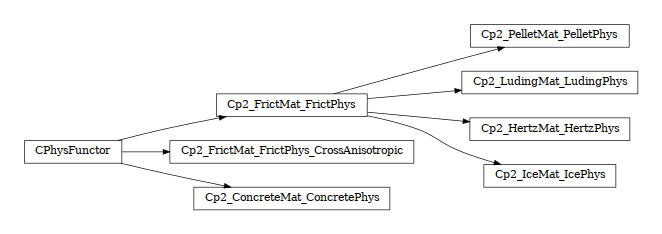class woo.dem.CPhysFunctor(*args, **kwargs)

Functor for creating/updating Contact.phys objects.

1. __init__(self: woo.dem.CPhysFunctor) -> None

2. __init__(self: woo.dem.CPhysFunctor, *args, **kwargs) -> None

##### Cp2_FrictMat_FrictPhys_CrossAnisotropic¶
class woo.dem.Cp2_FrictMat_FrictPhys_CrossAnisotropic(*args, **kwargs)

Call Cp2_FrictMat_FrictPhys to create a new FrictPhys, but multiply resulting normal and shear stiffnesses by smooth dimensionless anisotropy distribution given by alpha and beta. The functionality is demonstrated in the following movie:

1. __init__(self: woo.dem.Cp2_FrictMat_FrictPhys_CrossAnisotropic) -> None

2. __init__(self: woo.dem.Cp2_FrictMat_FrictPhys_CrossAnisotropic, *args, **kwargs) -> None

E1(= 1000000.0)

In-plane normal modulus

[type: Real, unit: Pa]

E2(= 100000.0)

Out-of-plane normal modulus

[type: Real, unit: Pa]

G1(= 10000.0)

In-plane shear modulus

[type: Real, unit: Pa]

G2(= 10000.0)

Out-of-plane shear modulus

[type: Real, unit: Pa]

nu1(= 0.4)

Major Poisson’s ratio; dependent value computed as $$\frac{E_1}{2G_1}-1$$.

alpha(= 0.0)

Strike angle for the local axes

[type: Real, unit: rad, range: 0−6.28319]

beta(= 0.0)

Dip angle for the local axes

[type: Real, unit: rad, range: 0−1.5708]

xisoAxis(= Vector3(1, 0, 0))

Axis (normal) of the cross-anisotropy in global coordinates; computed from alpha and beta as $$\vec{n}=(\cos\alpha\sin\beta,-\sin\alpha\sin\beta,\cos\beta)$$.

recomputeStep(= -1)

Keep track of updates to alpha and beta, so that stiffnesses of existing contacts are forced to be updated.

[type: int]

##### Cp2_ConcreteMat_ConcretePhys¶
class woo.dem.Cp2_ConcreteMat_ConcretePhys(*args, **kwargs)

Compute ConcretePhys from two ConcreteMat instances. Uses simple (arithmetic) averages if material are different. Simple copy of parameters is performed if the instance of ConcreteMat is shared.

1. __init__(self: woo.dem.Cp2_ConcreteMat_ConcretePhys) -> None

2. __init__(self: woo.dem.Cp2_ConcreteMat_ConcretePhys, *args, **kwargs) -> None

cohesiveThresholdStep(= 10)

Should new contacts be cohesive? They will before this iter#, they will not be afterwards. If 0, they will never be. If negative, they will always be created as cohesive (10 by default).

[type: long]

##### Cp2_FrictMat_FrictPhys¶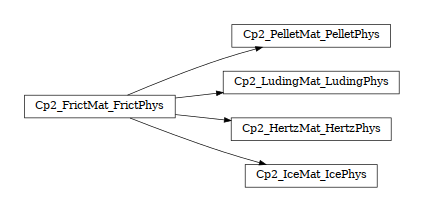class woo.dem.Cp2_FrictMat_FrictPhys(*args, **kwargs)

TODO

1. __init__(self: woo.dem.Cp2_FrictMat_FrictPhys) -> None

2. __init__(self: woo.dem.Cp2_FrictMat_FrictPhys, *args, **kwargs) -> None

tanPhi(= None)

Instance of MatchMaker determining how to compute contact friction angle. If None, minimum value is used.

[type: shared_ptr<MatchMaker>]

###### Cp2_LudingMat_LudingPhys¶
class woo.dem.Cp2_LudingMat_LudingPhys(*args, **kwargs)

Compute LudingPhys given two instances of LudingMat.

1. __init__(self: woo.dem.Cp2_LudingMat_LudingPhys) -> None

2. __init__(self: woo.dem.Cp2_LudingMat_LudingPhys, *args, **kwargs) -> None

###### Cp2_IceMat_IcePhys¶
class woo.dem.Cp2_IceMat_IcePhys(*args, **kwargs)

Compute IcePhys given two instances of :refIceMat.

1. __init__(self: woo.dem.Cp2_IceMat_IcePhys) -> None

2. __init__(self: woo.dem.Cp2_IceMat_IcePhys, *args, **kwargs) -> None

bonds0(= 0)

Bonding bits for new contacts, for the initial configuration.

[type: int, bit accessors: bondN, bondT, bondW, bondR, brkN, brkT, brkW, brkR]

bonds1(= 0)

Bonding bits for new contacts, for contacts created after the initial configuration.

[type: int, bit accessors: bondN, bondT, bondW, bondR, brkN, brkT, brkW, brkR]

step01(= 3)

Step after which bonds1 will be used instead of bonds0 for new contacts.

[type: int]

###### Cp2_HertzMat_HertzPhys¶
class woo.dem.Cp2_HertzMat_HertzPhys(*args, **kwargs)

Compute HertzPhys given two instances of :refHertzMat. The value of HertzPhys.alpha is averaged (from HertzMat.alpha) while HertzPhys.gamma is minimum (of HertzMat.gamma).

1. __init__(self: woo.dem.Cp2_HertzMat_HertzPhys) -> None

2. __init__(self: woo.dem.Cp2_HertzMat_HertzPhys, *args, **kwargs) -> None

poisson(= 0.2)

Poisson ratio for computing contact properties (not provided by the material class currently)

[type: Real]

en(= nan)

Normal coefficient of restitution (if outside the 0-1 range, there will be no damping, making en effectively equal to one).

[type: Real]

###### Cp2_PelletMat_PelletPhys¶
class woo.dem.Cp2_PelletMat_PelletPhys(*args, **kwargs)

Compute PelletPhys given two instances of :refPelletMat. PelletMat.normPlastCoeff is averaged into PelletPhys.normPlastCoeff, while minimum of PelletMat.kaDivKn is taken to compute PelletPhys.ka.

1. __init__(self: woo.dem.Cp2_PelletMat_PelletPhys) -> None

2. __init__(self: woo.dem.Cp2_PelletMat_PelletPhys, *args, **kwargs) -> None

#### CPhysDispatcher¶

class woo.dem.CPhysDispatcher(*args, **kwargs)

Dispatcher calling functors based on received argument type(s).

1. __init__(self: woo.dem.CPhysDispatcher) -> None

2. __init__(self: woo.dem.CPhysDispatcher, *args, **kwargs) -> None

functors(= [])

Functors active in the dispatch mechanism [overridden below].

[type: vector<shared_ptr<CPhysFunctor>>]

dispFunctor(self: woo.dem.CPhysDispatcher, arg0: woo.dem.Material, arg1: woo.dem.Material)woo.dem.CPhysFunctor

Return functor that would be dispatched for given argument(s); None if no dispatch; ambiguous dispatch throws.

dispMatrix(self: woo.dem.CPhysDispatcher, names: bool = True)dict

Return dictionary with contents of the dispatch matrix.

### Contact law¶

TODO

#### CData¶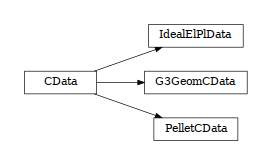class woo.dem.CData(*args, **kwargs)

Optional data stored in the contact by the Law functor.

1. __init__(self: woo.dem.CData) -> None

2. __init__(self: woo.dem.CData, *args, **kwargs) -> None

#### G3GeomCData¶

class woo.dem.G3GeomCData(*args, **kwargs)

Internal variables for use with G3Geom

1. __init__(self: woo.dem.G3GeomCData) -> None

2. __init__(self: woo.dem.G3GeomCData, *args, **kwargs) -> None

shearForce(= Vector3(0, 0, 0))

Shear force in global coordinates

[type: Vector3r]

#### PelletCData¶

class woo.dem.PelletCData(*args, **kwargs)

Hold state variables for pellet contact.

1. __init__(self: woo.dem.PelletCData) -> None

2. __init__(self: woo.dem.PelletCData, *args, **kwargs) -> None

uNPl(= 0.0)

Plastic displacement on the contact.

[type: Real]

uN0(= 0.0)

Initial distance (defines equilibrium).

[type: Real]

#### IdealElPlData¶

class woo.dem.IdealElPlData(*args, **kwargs)

Hold (optional) state variables for ideally elasto-plastic contacts.

1. __init__(self: woo.dem.IdealElPlData) -> None

2. __init__(self: woo.dem.IdealElPlData, *args, **kwargs) -> None

uN0(= 0.0)

Reference (equilibrium) value for uN (normal displacement).

[type: Real]

#### LawFunctor¶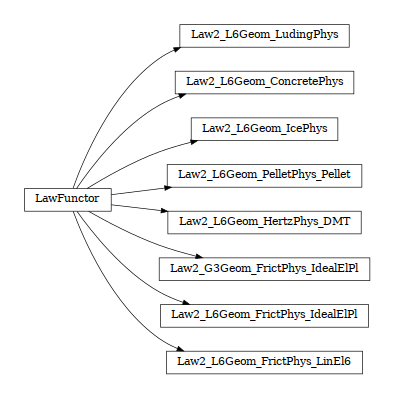class woo.dem.LawFunctor(*args, **kwargs)

Functor for applying constitutive laws on contact.

1. __init__(self: woo.dem.LawFunctor) -> None

2. __init__(self: woo.dem.LawFunctor, *args, **kwargs) -> None

##### Law2_L6Geom_IcePhys¶
class woo.dem.Law2_L6Geom_IcePhys(*args, **kwargs)

Contact law implementing ice-contact-model.

1. __init__(self: woo.dem.Law2_L6Geom_IcePhys) -> None

2. __init__(self: woo.dem.Law2_L6Geom_IcePhys, *args, **kwargs) -> None

iniEqlb(= True)

Set the intial distance as equilibrium distance (saved in IcePhys.uN0, subtracted from L6Geom.uN); enabling during simulation will only affect newly created contacts).

[type: bool]

elastIx(= -1)

Index of elastic energy (cache).

brokenIx(= -1)

Index of energy which disappeared when contacts broke (cache).

plastIx(= -1)

Index of plastically dissipated energy (cache).

##### Law2_L6Geom_ConcretePhys¶
class woo.dem.Law2_L6Geom_ConcretePhys(*args, **kwargs)

Constitutive law for concrete.

1. __init__(self: woo.dem.Law2_L6Geom_ConcretePhys) -> None

2. __init__(self: woo.dem.Law2_L6Geom_ConcretePhys, *args, **kwargs) -> None

yieldSurfType(= 3)

yield function: 0: mohr-coulomb (original); 1: parabolic; 2: logarithmic, 3: log+lin_tension, 4: elliptic, 5: elliptic+log

[type: int, named enum, possible values are: ‘linear’ (‘lin’, ‘MC’, ‘mc’, ‘Mohr-Coulomb’; 0), ‘para’ (‘parabolic’; 1), ‘log’ (‘logarithmic’; 2), ‘log+lin’ (‘logarithmic, linear tension’, ‘loglin’; 3), ‘elliptic’ (‘ell’; 4), ‘elliptic+logarithmic’ (‘ell+log’; 5)]

yieldLogSpeed(= 0.1)

scaling in the logarithmic yield surface (should be <1 for realistic results; >=0 for meaningful results)

[type: Real]

yieldEllipseShift(= nan)

horizontal scaling of the ellipse (shifts on the +x axis as interactions with +y are given)

[type: Real]

omegaThreshold(= 1.0)

damage after which the contact disappears (<1), since omega reaches 1 only for strain →+∞

[type: Real]

epsSoft(= -0.003)

Strain at which softening in compression starts (non-negative to deactivate)

[type: Real]

relKnSoft(= 0.3)

Relative rigidity of the softening branch in compression (0=perfect elastic-plastic, <0 softening, >0 hardening)

[type: Real]

elastPotIx(= -1)

Index for elastic potential energy

[type: int, not saved, not accessible from python]

yieldSigmaTNorm(self: woo.dem.Law2_L6Geom_ConcretePhys, sigmaN: float, omega: float, coh0: float, tanPhi: float)float

Return radius of yield surface for given material and state parameters; uses attributes of the current instance (yieldSurfType etc), change them before calling if you need that.

##### Law2_L6Geom_FrictPhys_LinEl6¶
class woo.dem.Law2_L6Geom_FrictPhys_LinEl6(*args, **kwargs)

Ideally elastic-plastic behavior.

1. __init__(self: woo.dem.Law2_L6Geom_FrictPhys_LinEl6) -> None

2. __init__(self: woo.dem.Law2_L6Geom_FrictPhys_LinEl6, *args, **kwargs) -> None

charLen(= -1.0)

Characteristic length, which is equal to stiffnesses ratio kNormal/kTwist and kShear/kBend. Must be non-negative.

[type: Real, unit: m]

elastPotIx(= -1)

Index for elastic potential energy

[type: int, not saved, not accessible from python]

##### Law2_L6Geom_HertzPhys_DMT¶
class woo.dem.Law2_L6Geom_HertzPhys_DMT(*args, **kwargs)

Law for Hertz contact with optional adhesion (DMT (Derjaguin-Muller-Toporov) [DMT75]), non-linear viscosity ([AE11]) The formulation is taken mainly from [Joh87]. The parameters are given through Cp2_FrictMat_HertzPhys. More details are given in hertzian_contact_models.

1. __init__(self: woo.dem.Law2_L6Geom_HertzPhys_DMT) -> None

2. __init__(self: woo.dem.Law2_L6Geom_HertzPhys_DMT, *args, **kwargs) -> None

noAttraction(= True)

Avoid non-physical normal attraction which may result from viscous effects by making the normal force zero if there is attraction ($$F_n>0$$). This condition is only applied to elastic and viscous part of the normal force. Adhesion, if present, is not limited. See [AE11], the ‘Model choice’ section (pg. 5), for discussion of this effect. .. note:: It is technically not possible to break the contact completely while there is still geometrical overlap, so only force is set to zero but the contact still exists.

[type: bool]

nCallsIters

Count number of calls of the functor and of iterations in the Halley solver (if used).

[type: OpenMPArrayAccumulator<int>, not shown in the UI, not dumped]

avgIter(= nan)

Average number of Halley iterations per contact when using the Schwarz model (updated on-demand).

digits(= 26)

Precision for Halley iteration with the Schwarz model, measured in binary digits; the maximum is the number of digits of the floating point type for given platform. Precision above 2/3 of the maximum will very likely have no effect on the result, but it will require extra (few) iterations before converging.

[type: int, range: 1−53]

plastIx(= -1)

Index of plastically dissipated energy.

[type: int, not saved, not accessible from python]

viscNIx(= -1)

Index of viscous dissipation in the normal sense.

[type: int, not saved, not accessible from python]

viscTIx(= -1)

Index of viscous dissipation in the tangent sense.

[type: int, not saved, not accessible from python]

elastPotIx(= -1)

Index for elastic potential energy.

[type: int, not saved, not accessible from python]

dmtIx(= -1)

Index for elastic energy of new/broken contacts.

[type: int, not saved, not accessible from python]

resetCounters(self: woo.dem.Law2_L6Geom_HertzPhys_DMT)None

Reset nCallsIters and thus avgIter.

##### Law2_L6Geom_PelletPhys_Pellet¶
class woo.dem.Law2_L6Geom_PelletPhys_Pellet(*args, **kwargs)

Contact law with friction and plasticity in compression, designed for pellet behavior. See pellet-contact-model for details.

1. __init__(self: woo.dem.Law2_L6Geom_PelletPhys_Pellet) -> None

2. __init__(self: woo.dem.Law2_L6Geom_PelletPhys_Pellet, *args, **kwargs) -> None

thinRate(= 0.0)

The amount of reducing particle radius ($$\theta_t$$), relative to plastic deformation increment (non-positive to disable thinning)

[type: Real]

thinRelRMin(= 0.7)

Minimum radius reachable with sphere thinning at plastic deformation, relative to initial particle size ($$r_{\min}^{\mathrm{rel}}$$)

[type: Real]

thinExp(= -1.0)

Exponent for reducing the rate of thinning as the minimum radius is being approached ($$\gamma_t$$)

[type: Real]

thinRefRad(= 0.0)

Reference radius for thinning; if positive, thinRateExp and thinMinExp are in effect.

[type: Real, unit: m]

thinMinExp(= 0.0)

Multiply minimum radius $$r_0$$ by $$\left(\frac{r}{r_{\rm thinRefRad}}\right)^{\rm thinMinExp}$$ (size-dependent minimum radius).

[type: Real]

thinRateExp(= 0.0)

Multiply thinning rate $$\theta_t$$ (thinRate) by $$\left(\frac{r}{r_{\rm thinRefRad}}\right)^{\rm thinRateExp}$$ (size-dependent minimum radius).

[type: Real]

confSigma(= 0.0)

Confinement stress (acting on contact area). Negative values will make particles stick together. The strain-stress diagram is shifted vertically with this parameter. The value of confinement can be further scaled with confRefRad.

Note

Energy computation might be incorrect with confinement (not yet checked).

[type: Real]

confRefRad(= 0.0)

If positive, scale the confining stress (confSigma) using the value of $$\left(\frac{A}{\pi r_{\rm ref}^2}\right)^{\beta_c}$$; this allows to introduce confinement which varies depending on particle size.

[type: Real, unit: m]

confExp(= 1.0)

Dimensionless exponent to be used in conjunction with confRefRad.

[type: Real]

iniEqlb(= False)

Use the initial distance as equilibrium.

[type: bool]

plastSplit(= False)

Track energy dissipated in normal and tangential sliding separately

[type: bool]

plastIx(= -1)

Index of plastically dissipated energy

[type: int, not saved, not accessible from python]

normPlastIx(= -1)

Index of plastically dissipated energy in the normal sense

[type: int, not saved, not accessible from python]

elastPotIx(= -1)

Index for elastic potential energy

[type: int, not saved, not accessible from python]

static adhesionForce(uN: float, uNPl: float, ka: float)float

Adhesion force function $h$ evaluated with given parameters

static yieldForce(uN: float, d0: float, kn: float, alpha: float)float

Return yield force for alpha and given parameters.

static yieldForceDerivative(uN: float, d0: float, kn: float, alpha: float)float

Return yield force derivative for given parameters.

##### Law2_G3Geom_FrictPhys_IdealElPl¶
class woo.dem.Law2_G3Geom_FrictPhys_IdealElPl(*args, **kwargs)

Ideally elastic-plastic behavior, for use with G3Geom.

1. __init__(self: woo.dem.Law2_G3Geom_FrictPhys_IdealElPl) -> None

2. __init__(self: woo.dem.Law2_G3Geom_FrictPhys_IdealElPl, *args, **kwargs) -> None

noSlip(= False)

Disable plastic slipping

[type: bool]

noBreak(= False)

Disable removal of contacts when in tension.

[type: bool]

plastDissipIx(= -1)

Index of plastically dissipated energy

[type: int, not saved, not accessible from python]

elastPotIx(= -1)

Index for elastic potential energy

[type: int, not saved, not accessible from python]

watch(= Vector2i(-1, -1))

Print debug information for this couple of IDs

[type: Vector2i]

##### Law2_L6Geom_FrictPhys_IdealElPl¶
class woo.dem.Law2_L6Geom_FrictPhys_IdealElPl(*args, **kwargs)

Ideally elastic-plastic behavior.

1. __init__(self: woo.dem.Law2_L6Geom_FrictPhys_IdealElPl) -> None

2. __init__(self: woo.dem.Law2_L6Geom_FrictPhys_IdealElPl, *args, **kwargs) -> None

iniEqlb(= False)

Consider the intial distance as equilibrium distance (saved in contact data, subtracted from L6Geom.uN); enabling during simulation will only affect newly created contacts; disabling will affect all contacts.

[type: bool]

relRollStiff(= 0.0)

Rolling stiffness relative to FrictPhys.kn × charLen (with wcharLen being the sum of L6Geom.lens). If non-positive, there is no rolling/twisting resistance.

[type: Real]

relTwistStiff(= 0.0)

Twisting stiffness relative to rolling stiffness (see relRollStiff).

[type: Real]

rollTanPhi(= 0.0)

Rolling friction angle – the rolling force will not exceed Fn × rollTanPhi. This value is applied separately to twisting as well. If non-positive, there is no rolling/twisting resistance.

[type: Real, range: 0−1.5708]

noSlip(= False)

Disable plastic slipping

[type: bool]

noBreak(= False)

Disable removal of contacts when in tension.

[type: bool]

noFrict(= False)

Turn off friction computation, it will be always zero regardless of material parameters

[type: bool]

plastDissipIx(= -1)

Index of plastically dissipated energy

[type: int, not saved, not accessible from python]

elastPotIx(= -1)

Index for elastic potential energy

[type: int, not saved, not accessible from python]

brokenIx(= -1)

Index for energy lost in broken contacts with non-zero force

[type: int, not saved, not accessible from python]

##### Law2_L6Geom_LudingPhys¶
class woo.dem.Law2_L6Geom_LudingPhys(*args, **kwargs)

Contact law implementing luding-contact-model.

1. __init__(self: woo.dem.Law2_L6Geom_LudingPhys) -> None

2. __init__(self: woo.dem.Law2_L6Geom_LudingPhys, *args, **kwargs) -> None

wImmediate(= True)

Increment plastic & viscous work in S.energy in every step rather than commiting the sum when contact dissolves. Note that Particle.matState (if a LudingMatState) is always updated only when the contact dissolves.

[type: bool]

viscIx(= -1)

Index of viscous dissipation.

[type: int, not saved, not accessible from python]

plastIx(= -1)

Index of plastic dissipation.

[type: int, not saved, not accessible from python]

#### LawDispatcher¶

class woo.dem.LawDispatcher(*args, **kwargs)

Dispatcher calling functors based on received argument type(s).

1. __init__(self: woo.dem.LawDispatcher) -> None

2. __init__(self: woo.dem.LawDispatcher, *args, **kwargs) -> None

functors(= [])

Functors active in the dispatch mechanism [overridden below].

[type: vector<shared_ptr<LawFunctor>>]

dispFunctor(self: woo.dem.LawDispatcher, arg0: woo.dem.CGeom, arg1: woo.dem.CPhys)woo.dem.LawFunctor

Return functor that would be dispatched for given argument(s); None if no dispatch; ambiguous dispatch throws.

dispMatrix(self: woo.dem.LawDispatcher, names: bool = True)dict

Return dictionary with contents of the dispatch matrix.

#### Testing contact laws¶

TODO

##### LawTester¶
class woo.dem.LawTester(*args, **kwargs)

Engine for testing contact laws by prescribing various loading scenarios, which are a combination of prescribing force or velocity along given contact-local axes.

1. __init__(self: woo.dem.LawTester) -> None

2. __init__(self: woo.dem.LawTester, *args, **kwargs) -> None

ids(= Vector2i(5923313, 0))

Ids of particles in contact

[type: Vector2i]

done(= 'tester.dead=True')

Python expression to run once all stages had finished. This is run after LawTesterStage.done of the last stage.

[type: string]

abWeight(= 1.0)

Float, usually ∈〈0,1〉, determining on how are displacements/rotations distributed between particles (0 for A, 1 for B); intermediate values will apply respective part to each of them.

[type: Real]

f(= Vector6(0, 0, 0, 0, 0, 0))

Force on contact, NaN if contact is broken

k(= Vector6(0, 0, 0, 0, 0, 0))

Tangent contact stiffness, NaN if there is no contact (or the contact model does not define it). Diagonal of the K matrix in df=Kdu.

smooF(= Vector6(0, 0, 0, 0, 0, 0))

Smoothed value of generalized contact forces.

u(= Vector6(0, 0, 0, 0, 0, 0))

Cumulative value of contact displacement, NaN if contact is broken

smooU(= Vector6(0, 0, 0, 0, 0, 0))

Smoothed value of generalized contact displacements.

v(= Vector6(0, 0, 0, 0, 0, 0))

Relative velocity on contact; NaN if the contact is broken

smooV(= Vector6(0, 0, 0, 0, 0, 0))

Smoothed value of generalized contact relative velocity.

fErrRel(= Vector6(inf, inf, inf, inf, inf, inf))

Relative error of contact force (with respect to smoothed value)

fErrAbs(= Vector6(inf, inf, inf, inf, inf, inf))

Absolute error of contact force (with respect to smoothed value)

uErrRel(= Vector6(inf, inf, inf, inf, inf, inf))

Relative error of contact displacement (with respect to smoothed value)

uErrAbs(= Vector6(inf, inf, inf, inf, inf, inf))

Absolute error of contact displacement (with respect to smoothed value)

vErrRel(= Vector6(inf, inf, inf, inf, inf, inf))

Relative error of contact velocity (with respect to smoothed value)

vErrAbs(= Vector6(inf, inf, inf, inf, inf, inf))

Absolute error of contact velocity (with respect to smoothed value)

smoothErr(= -1.0)

Smoothing factor for computing errors; if negative, set to smooth automatically.

[type: Real]

smooth(= 0.0001)

Smoothing factor for computing smoothF

[type: Real]

stage(= 0)

Current stage to be finished

stageT0(= -1.0)

Time at which this stage was entered

stages(= [])

Stages to be reached during the testing

[type: vector<shared_ptr<LawTesterStage>>]

maxStageSteps(= 100000)

Throw error if stage takes this many steps

[type: int, not shown in the UI]

fuv(self: woo.dem.LawTester)dict

Return python dictionary containing f,u,v,smooF,smooU,smooU; useful for plotting with woo.plot.addData(**tester.fuv())

restart(self: woo.dem.LawTester)None

Reset the tester to initial state; all stages are reset via LawTesterStage.reset, the woo.core.Engine.dead flag is unset.

##### LawTesterStage¶
class woo.dem.LawTesterStage(*args, **kwargs)

Stage to be reached by LawTester.

1. __init__(self: woo.dem.LawTesterStage) -> None

2. __init__(self: woo.dem.LawTesterStage, *args, **kwargs) -> None

values(= Vector6(0, 0, 0, 0, 0, 0))

Prescribed values during this step (DoF-ordering: x,y,z linear, x,y,z angular)

[type: Vector6r]

whats(= Vector6i(0, 0, 0, 0, 0, 0))

Meaning of values components (DoF-odering). The constructor acceps this attribute specified as string of 6 characters, where each of them can be: . for imposing nothing, v for imposing velocity, i for imposing initial velocity only, f for imposing force.

[type: Vector6i]

until(= '')

Stage finishes when until (python expression) evaluates to True. Besides receiving global variables, several local variables are passed: C (contact object; None if contact does not exist), pA (first particle), pB (second particle), scene (current scene object), tester (LawTester object), stage (LawTesterStage object).

[type: string]

untilEvery(= 1)

Test the until expression only every untilEvery steps (this may make the execution faster)

[type: int]

done(= '')

Run this python command when the stage finishes

[type: string]

step(= 0)

Step in this stage

[type: int]

time(= 0.0)

Time in this stage

[type: Real]

hadC(= False)

Flag keeping track of whether there was a contact in this stage at all

[type: bool]

hasC(= False)

Flag keeping track of whether there was a contact in this stage at all

[type: bool]

timeC0(= nan)

Time of creating of the last contact (NaN if there has never been one).

[type: Real]

bounces(= 0)

Number of sign changes of the normal relative velocity in this stage

[type: int]

property broken

Test whether an existing contact broke in this stage; this is useful for saying until='stage.broken' (equivalent to stage.hadC and not stage.hasC). This is different from until='not C', since this condition will be satisfied before any contact exists at all.

property cTime

Time since creation of the last contact (NaN if there has never been one). Useful for testing collision time after the condition until='stage.rebound' has been satisfied. Equivalent to stage.time-stage.timeC0.

property rebound

Test for rebound; rebound is considered complete when sign of relative normal velocity changed more than once (adhesive contacts may never separate once they are created – this catches a single period of the oscillation) or if contact breaks. Equivalent to stage.bounces>=2 or stage.broken.

reset(self: woo.dem.LawTesterStage)None

Reset this stage to its initial stage such that it can be used again as if new. This is called automatically from LawTester.restart.

## HalfspaceBuoyancy¶

class woo.dem.HalfspaceBuoyancy(*args, **kwargs)

Apply Buoyancy force for uninodal particles, depending on their position in the $$-z$$ half-space in local coordinate system (node). TODO: references to papers, wikipedia with formulas, or write formulas here.

1. __init__(self: woo.dem.HalfspaceBuoyancy) -> None

2. __init__(self: woo.dem.HalfspaceBuoyancy, *args, **kwargs) -> None

mask(= 5)

Mask for particles Buoyancy is applied to.

[type: uint]

liqRho(= 1000.0)

Density of the medium.

[type: Real]

dragCoef(= 0.47)

Drag coefficient.

[type: Real]

drag(= False)

Flag for turning water drag on or off

[type: bool]

surfPt(= Vector3(0, 0, 0))

Point on liquid surface; the surface is perpendicular to :obj:woo.dem.DemField.gravity (which must not be zero).

[type: Vector3r]

## BoxTraceTimeSetter¶

class woo.dem.BoxTraceTimeSetter(*args, **kwargs)

Set TraceVisRep.t0 of nodes inside box to the current time <Scene.time>; this is used for tracking how long has a particle been away from that region.

1. __init__(self: woo.dem.BoxTraceTimeSetter) -> None

2. __init__(self: woo.dem.BoxTraceTimeSetter, *args, **kwargs) -> None

node(= None)

Optionally define local coordinate system for box.

[type: shared_ptr<Node>]

box(= AlignedBox3((1.7976931348623157e308, 1.7976931348623157e308, 1.7976931348623157e308), (-1.7976931348623157e308, -1.7976931348623157e308, -1.7976931348623157e308)))

Box volume; in local coordinates if node is given, global otherwise.

[type: AlignedBox3r]

glColor(= 0.5)

Color for rendering (NaN disables), mapped using the default colormap.

[type: Real]

## MeshVolume¶

class woo.dem.MeshVolume(*args, **kwargs)

Compute volume of (possibly deforming) closed triangulated surface; depends on the gts feature.

1. __init__(self: woo.dem.MeshVolume) -> None

2. __init__(self: woo.dem.MeshVolume, *args, **kwargs) -> None

mask(= 0)

[type: int]

reinit(= False)

If true, recreate internal data from scratch

[type: bool]

nodes(= NodeList[])

List of nodes, in the same order as the GTS surface structure.

[type: vector<shared_ptr<Node>>, not shown in the UI, not saved]

vol(= nan)

Volume as computed when last run

[type: Real]

thickVol(= nan)

Volume of the inner side of the mesh: the mesh is defined by facets' midplanes, but some facets may have non-zero Facet.halfThick. This number is the sum of (initial!) facet area times Facet.halfThick. To get the volume with this part subtracted, use netVol.

[type: Real]

property netVol

Net volume: volume minus thickVol.

## Motion integration¶

TODO

### Leapfrog¶

class woo.dem.Leapfrog(*args, **kwargs)

Engine integrating newtonian motion equations, using the leap-frog scheme. See theory-motion-integration for details.

1. __init__(self: woo.dem.Leapfrog) -> None

2. __init__(self: woo.dem.Leapfrog, *args, **kwargs) -> None

IpLL4h(= Matrix3(0, 0, 0, 0, 0, 0, 0, 0, 0))

$$I+\frac{\nnext{\tens{L}}+\pprev{\tens{L}}}{4}\Dt$$

[type: Matrix3r, not saved, read-only in python]

ImLL4hInv(= Matrix3(0, 0, 0, 0, 0, 0, 0, 0, 0))

$$\left(\tens{I}-\frac{\nnext{\tens{L}}-\pprev{\tens{L}}}{4}\Dt\right)^{-1}$$

[type: Matrix3r, not saved, read-only in python]

LmL(= Matrix3(0, 0, 0, 0, 0, 0, 0, 0, 0))

$$\nnext{\tens{L}}-\pprev{\tens{L}}$$

[type: Matrix3r, not saved, read-only in python]

deltaSpinVec(= Vector3(0, 0, 0))

$$-\frac{1}{2}\epsilon_{ijk}\frac{\pprev{\tens{L}}-{\pprev{\tens{L}}}^T}{2}+\frac{1}{2}\epsilon_{ijk}\frac{\nnext{\tens{L}}-{\nnext{\tens{L}}}^T}{2}$$.

[type: Vector3r, not saved, read-only in python]

damping(= 0.2)

damping coefficient for non-viscous damping

[type: Real]

reset(= False)

Reset forces immediately after applying them.

[type: bool]

_forceResetChecked(= False)

Whether we already issued a warning for forces being (probably) not reset

[type: bool, not shown in the UI]

maxVelocitySq(= nan)

store square of max. velocity, for informative purposes; computed again at every step.

dontCollect(= False)

Don’t attempt to collect DEM nodes when there are none in the first step.

[type: bool, not shown in the UI]

kinSplit(= False)

Whether to separately track translational and rotational kinetic energy.

[type: bool]

nonviscDampIx(= -1)

Index of the energy dissipated using the non-viscous damping (damping).

[type: int, not saved, not accessible from python]

gravWorkIx(= -1)

Index for gravity work

[type: int, not saved, not accessible from python]

kinEnergyIx(= -1)

Index for kinetic energy in scene->energies.

[type: int, not saved, not accessible from python]

kinEnergyTransIx(= -1)

Index for translational kinetic energy in scene->energies.

[type: int, not saved, not accessible from python]

kinEnergyRotIx(= -1)

Index for rotational kinetic energy in scene->energies.

[type: int, not saved, not accessible from python]

### ForceResetter¶

class woo.dem.ForceResetter(*args, **kwargs)

Reset forces on nodes in DEM field.

1. __init__(self: woo.dem.ForceResetter) -> None

2. __init__(self: woo.dem.ForceResetter, *args, **kwargs) -> None

### DynDt¶

class woo.dem.DynDt(*args, **kwargs)

Adjusts Scene.dt based on current stiffness of particle contacts.

1. __init__(self: woo.dem.DynDt) -> None

2. __init__(self: woo.dem.DynDt, *args, **kwargs) -> None

maxRelInc(= 0.0001)

Maximum relative increment of timestep within one step, to void abrupt changes in timestep leading to numerical artefacts.

[type: Real]

dryRun(= False)

Only set dt to the value of timestep, don’t apply it really.

[type: bool]

dt(= nan)

New timestep value which would be used if dryRun were not set. Unused when dryRun is false.

[type: Real]

nodalCritDtSq(self: woo.dem.DynDt, node: woo.core.Node)float

### DemData¶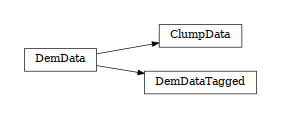class woo.dem.DemData(*args, **kwargs)

Dynamic state of node.

1. __init__(self: woo.dem.DemData) -> None

2. __init__(self: woo.dem.DemData, *args, **kwargs) -> None

vel(= Vector3(0, 0, 0))

Linear velocity.

[type: Vector3r, unit: m/s]

angVel(= Vector3(0, 0, 0))

Angular velocity; when set, angMom is reset (and updated from angVel in Leapfrog)..

mass(= 0.0)

Associated mass.

[type: Real, unit: kg]

inertia(= Vector3(0, 0, 0))

Inertia along local (principal) axes

[type: Vector3r, unit: kg·m²]

force(= Vector3(0, 0, 0))

Applied force

[type: Vector3r, unit: N]

torque(= Vector3(0, 0, 0))

Applied torque

[type: Vector3r, unit: N·m]

angMom(= Vector3(nan, nan, nan))

Angular momentum; used with the aspherical integrator. If NaN and aspherical integrator (Leapfrog) is used, the value is initialized to inertia × angVel.

[type: Vector3r, unit: N·m·s]

flags(= 0)

Bit flags storing blocked DOFs, clump status, …

[type: unsigned, read-only in python, bit accessors: blockX, blockY, blockZ, blockRotX, blockRotY, blockRotZ, clumped, clump, energySkip, gravitySkip, tracerSkip, dampingSkip]

linIx(= -1)

Index within DemField.nodes (for efficient removal)

[type: long, not shown in the UI, read-only in python]

parRef(= [])

Back-reference for particles using this node; this is important for knowing when a node may be deleted (no particles referenced) and such. Should be kept consistent.

[type: std::list<Particle*>, not shown in the UI, not saved, not accessible from python]

impose(= None)

Impose arbitrary velocity, angular velocity, … on the node; the functor is called from Leapfrog, after new position and velocity have been computed.

[type: shared_ptr<Impose>]

master

Master node; currently only used with clumps (since this is never set from python, it is safe to use weak_ptr).

[type: weak_ptr<Node>, not shown in the UI, not accessible from python]

addParRef(self: woo.dem.DemData, arg0: woo.dem.Particle)None
property blocked

Degress of freedom where linear/angular velocity will be always constant (equal to zero, or to an user-defined value), regardless of applied force/torque. String that may contain ‘xyzXYZ’ (translations and rotations).

guessMoving(self: woo.dem.DemData)bool

Tell whether the node is likely to be moving or not. Returns true if any of the following is true:

This function is used as heuristics by S.dem.par.add when called with nodes=-1 (default), to decide whether the particle’s nodes should be added to S.dem.nodes.

property isAspherical

Return True when inertia components are not equal.

static setOriMassInertia(arg0: woo.core.Node)None

Lump mass and inertia from all attached particles and attempt to rotate the node so that its axes are principal axes of inertia, if allowed by particles shape (without chaning the geometry).

property useAsphericalLeapfrog

Say whether the node will use aspherical leapfrog integration routine. The criterion is that the node is aspherical and does not have all rotations blocked.

#### ClumpData¶

class woo.dem.ClumpData(*args, **kwargs)

Data of a DEM particle which binds multiple particles together.

1. __init__(self: woo.dem.ClumpData) -> None

2. __init__(self: woo.dem.ClumpData, *args, **kwargs) -> None

nodes(= NodeList[])

Member nodes

[type: vector<shared_ptr<Node>>, not shown in the UI, read-only in python]

relPos(= [])

Relative member’s positions

relOri(= [])

Relative member’s orientations

equivRad(= nan)

Equivalent radius, for PSD statistics (e.g. in BoxOutlet).

[type: Real]

static applyToMembers(arg0: woo.core.Node)None

Move/rotate member nodes; this is only useful after setting clump’s node position/orientation by hand, so that memebrs are updated without running Leapfrog.

static forceTorqueFromMembers(arg0: woo.core.Node)tuple

Return the tuple (F,T), summary force and torque values collected from clump members, as acting on the clump node passed as argument.

#### DemDataTagged¶

class woo.dem.DemDataTagged(*args, **kwargs)

Add integer tag to each node, used with ForcesToHdf5 so that nodes can be numbered differently than in Woo.

1. __init__(self: woo.dem.DemDataTagged) -> None

2. __init__(self: woo.dem.DemDataTagged, *args, **kwargs) -> None

tag(= -1)

External identifier of th node (unused by Woo itself).

[type: int]

### Impose¶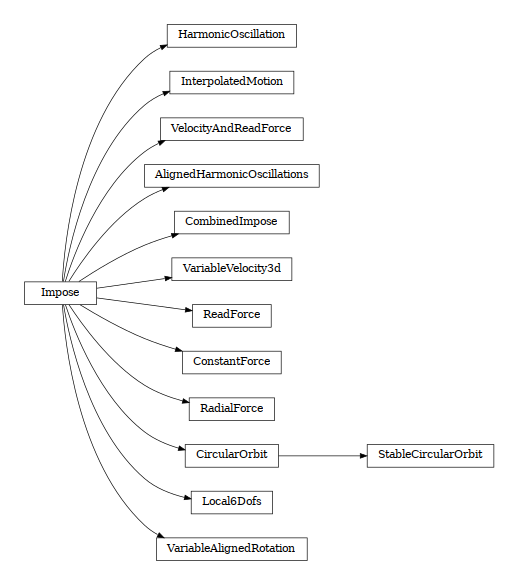class woo.dem.Impose(*args, **kwargs)

Impose arbitrary changes in Node and DemData, at certain hook points during motion integration. Velocity is imposed after motion integration (and again after computing acceleration from forces, to make sure forces don’t alter what is prescribed), force is imposed when forces from the previous step are being reset (thus additional force may be applied on the node as usual); readForce is a special imposition before resetting force, which is meant to look at summary force applied onto node(s).

1. __init__(self: woo.dem.Impose) -> None

2. __init__(self: woo.dem.Impose, *args, **kwargs) -> None

what(= 0)

What values are to be imposed; this is set by the derived engine automatically depending on what is to be prescribed.

[type: int, bit accessors: vel, force, iniVel, readForce]

stepLast(= -1)

Step in which this imposition was last used; updated atomically by callers from c++ by calling isFirstStepRun.

timeLast(= nan)

Time in which this imposition was last used; updated automatically bu callers from c++ via isFirstStepRun, just like stepLast.

__add__(self: woo.dem.Impose, arg0: woo.dem.Impose)woo.dem.Impose

Return combined imposition, a new instance of CombinedImpose.

#### CircularOrbit¶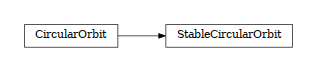class woo.dem.CircularOrbit(*args, **kwargs)

Imposes circular orbiting around the local z-axis; the velocity is prescribed using approximated midstep position in an incremental manner. This can lead to unstabilities (such as changing radius) when used over millions of steps, but does not require radius to be given explicitly (see also StableCircularOrbit). Angular velocity is touched only when rotate is set.

1. __init__(self: woo.dem.CircularOrbit) -> None

2. __init__(self: woo.dem.CircularOrbit, *args, **kwargs) -> None

node(= <Node @ 0x17c3330, at (0, 0, 0)>)

Local coordinate system.

[type: shared_ptr<Node>]

rotate(= False)

Impose rotational velocity so that orientation relative to the local z-axis is always the same. If false, angular velocity is left as-is.

[type: bool]

omega(= nan)

Orbiting angular velocity.

[type: Real]

angle(= 0.0)

Cumulative angle turned, incremented at every step.

[type: Real]

##### StableCircularOrbit¶
class woo.dem.StableCircularOrbit(*args, **kwargs)

Impose circular orbiting around local z-axis, enforcing constant radius of orbiting. The note about rotate applies to this imposition.

1. __init__(self: woo.dem.StableCircularOrbit) -> None

2. __init__(self: woo.dem.StableCircularOrbit, *args, **kwargs) -> None

radius(= nan)

Radius, i.e. enforced distance from the rotation axis.

[type: Real]

#### VariableVelocity3d¶

class woo.dem.VariableVelocity3d(*args, **kwargs)

Impose velocity in 3 independent senses, interpolated from piecewise-linear velocity function values, optional periodic in time; NaN values of velocity will impose nothing in that direction.

1. __init__(self: woo.dem.VariableVelocity3d) -> None

2. __init__(self: woo.dem.VariableVelocity3d, *args, **kwargs) -> None

times(= [])

Time values for vels.

[type: vector<Real>]

vels(= [])

Components of velocity, in local coordinates defined by ori.

[type: vector<Vector3r>]

angVels(= [])

Components of angular velocity, in local coordinates defined by ori. If empty, angular velocity will not be imposed at all.

Note

Only nodes which are using spherical integration can be prescribed angular velocity, since the aspherical integrator in woo.dem.Leapfrog uses angular momentum rather than angular velocity as input. This can be queried with Node.dem.useAsphericalLeapfrog.

[type: vector<Vector3r>]

ori(= Quaternion((1, 0, 0), 0))

Orientation of coordinate axes (by default, impose velocity along global axes)

[type: Quaternionr]

hooks(= [])

Python hooks to be run when time points are reached (max Δt later than times items). Must be same length than times, or shorter; use empty string for not doing anything at that time point.

[type: vector<string>]

diff(= False)

Prescribed velocity can be applied as total velocity value (with diff==False) or as difference added to the actual nodal velocity (with diff==True).

[type: bool]

wrap(= False)

Wrap time around the last time value (float modulo), if greater.

[type: bool]

t0(= 0.0)

Time offset which is subtracted from t (before wrapping, if wrap is used).

[type: Real]

#### AlignedHarmonicOscillations¶

class woo.dem.AlignedHarmonicOscillations(*args, **kwargs)

Imposes three independent harmonic oscillations along global coordinate system axes.

1. __init__(self: woo.dem.AlignedHarmonicOscillations) -> None

2. __init__(self: woo.dem.AlignedHarmonicOscillations, *args, **kwargs) -> None

freqs(= Vector3(nan, nan, nan))

Frequencies for individual axes. NaN value switches that axis off, the component will not be touched

[type: Vector3r]

amps(= Vector3(0, 0, 0))

Amplitudes along individual axes.

[type: Vector3r]

#### InterpolatedMotion¶

class woo.dem.InterpolatedMotion(*args, **kwargs)

Impose linear and angular velocity such that given positions and orientations are reached in at given time-points.

1. __init__(self: woo.dem.InterpolatedMotion) -> None

2. __init__(self: woo.dem.InterpolatedMotion, *args, **kwargs) -> None

poss(= [])

Positions which will be interpolated between.

[type: vector<Vector3r>]

oris(= [])

Orientations which will be interpolated between.

[type: vector<Quaternionr>]

times(= [])

Times at which given positions and orientations should be reached.

[type: vector<Real>]

t0(= 0.0)

Time offset to add to all time points.

[type: Real]

#### HarmonicOscillation¶

class woo.dem.HarmonicOscillation(*args, **kwargs)

Impose harmonic oscillation around initial center position, with given frequency freq ($$f$$) and amplitude amp ($$A$$), by prescribing velocity. Nodal velocity magnitude along dir is $$x'(t)=A\omega\cos(\omega(t-t_0))$$ (with $$\omega=2\pi f$$), which is the derivative of the harmonic motion equation $$x(t)=A\sin(\omega(t-t_0))$$. The motion starts in zero (for $$t_0=0$$); to reverse the direction, either reverse dir or assign $$t_0=\frac{1}{2f}=\frac{\pi}{\omega}$$.

1. __init__(self: woo.dem.HarmonicOscillation) -> None

2. __init__(self: woo.dem.HarmonicOscillation, *args, **kwargs) -> None

freq(= nan)

Frequence of oscillation

[type: Real]

amp(= nan)

Amplitude of oscillation

[type: Real]

dir(= Vector3(1, 0, 0))

Direcrtion of oscillation (normalized automatically)

[type: Vector3r]

t0(= 0.0)

Time when the oscillator is in the center position (phase)

[type: Real]

perpFree(= False)

If true, only velocity in the dir sense will be prescribed, velocity in the perpendicular sense will be preserved.

[type: bool]

#### CombinedImpose¶

class woo.dem.CombinedImpose(*args, **kwargs)

Combine several impositions and apply them one after another.

1. __init__(self: woo.dem.CombinedImpose) -> None

2. __init__(self: woo.dem.CombinedImpose, *args, **kwargs) -> None

imps(= [])

Impositions which are combined together, always called in this order.

[type: vector<shared_ptr<Impose>>]

class woo.dem.RadialForce(*args, **kwargs)

Impose constant force towards an axis in 3d.

2. __init__(self: woo.dem.RadialForce, *args, **kwargs) -> None

nodeA(= None)

First node defining the axis

[type: shared_ptr<Node>]

nodeB(= None)

Second node defining the axis

[type: shared_ptr<Node>]

F(= 0.0)

Magnitude of the force applied. Positive value means away from the axis given by nodeA and nodeB.

[type: Real]

#### VariableAlignedRotation¶

class woo.dem.VariableAlignedRotation(*args, **kwargs)

Impose piecewise-linear angular velocity along axis, based on the timeAngVel.

1. __init__(self: woo.dem.VariableAlignedRotation) -> None

2. __init__(self: woo.dem.VariableAlignedRotation, *args, **kwargs) -> None

axis(= 0)

Rotation axis.

[type: int]

timeAngVel(= [])

Angular velocity values in time. Time values must be increasing.

[type: vector<Vector2r>]

wrap(= False)

Wrap time around the last time value (float modulo), if greater.

[type: bool]

class woo.dem.ReadForce(*args, **kwargs)

Sum forces and torques acting on all nodes with this imposition; this imposition does not change the behavior of particles in any way.

2. __init__(self: woo.dem.ReadForce, *args, **kwargs) -> None

node(= None)

Reference CS for forces and torque (they are recomputed as if acting on this point); if not given, everything is summed in global CS.

[type: shared_ptr<Node>]

F

Summary force

[type: OpenMPAccumulator<Vector3r>, not saved, read-only in python]

T

Summary torque

[type: OpenMPAccumulator<Vector3r>, not saved, read-only in python]

#### ConstantForce¶

class woo.dem.ConstantForce(*args, **kwargs)

Impose constant force, which is added to other acting forces.

1. __init__(self: woo.dem.ConstantForce) -> None

2. __init__(self: woo.dem.ConstantForce, *args, **kwargs) -> None

F(= Vector3(0, 0, 0))

Applied force (in global coordinates)

[type: Vector3r]

#### Local6Dofs¶

class woo.dem.Local6Dofs(*args, **kwargs)

Impose force or velocity along all local 6 axes given by the trsf matrix.

1. __init__(self: woo.dem.Local6Dofs) -> None

2. __init__(self: woo.dem.Local6Dofs, *args, **kwargs) -> None

ori(= Quaternion((1, 0, 0), 0))

Local coordinates rotation

[type: Quaternionr]

values(= Vector6(0, 0, 0, 0, 0, 0))

Imposed values; their meaning depends on the whats vector

[type: Vector6r]

whats(= Vector6i(0, 0, 0, 0, 0, 0))

Meaning of values components: 0 for nothing imposed (i.e. zero force), 1 for velocity, 2 for force values

[type: Vector6i]

class woo.dem.VelocityAndReadForce(*args, **kwargs)

Impose velocity in one direction, and optionally read and sum force on all nodes with this imposition (force is not changed in any way). Velocity lateral to the imposition may be free or zero depending on latBlock.

2. __init__(self: woo.dem.VelocityAndReadForce, *args, **kwargs) -> None

dir(= Vector3(1, 0, 0))

Direction (automatically normalized) for prescribed velocity and force readings.

[type: Vector3r]

vel(= 0.0)

Prescribed velocity magnitude.

[type: Real]

latBlock(= True)

Whether lateral velocity (perpendicular to vel) is set to zero, or left free.

[type: bool]

sumF

Summary force on nodes with this imposition, in the direction of vel.

invF(= False)

Invert force value (so that it has the meaning of reactin rather than force exerted).

[type: bool]

dist(= 0.0)

Cumulative displacement of this imposition.

[type: Real]

energyName(= '')

If given, and trackEnergy is True, cumulate work done by this imposition under this name

[type: string]

workIx(= -1)

[type: int, not saved, not accessible from python]

### Tracer¶

class woo.dem.Tracer(*args, **kwargs)

Save trace of node’s movement

1. __init__(self: woo.dem.Tracer) -> None

2. __init__(self: woo.dem.Tracer, *args, **kwargs) -> None

num(= 50)

Number of positions to save (when creating new glyph)

[type: int]

compress(= 2)

Ratio by which history is compress when all data slots are filled; if 0, cycle and don’t compress.

[type: int]

compSkip(= 2)

Number of leading points to skip during compression; if negative, the value of compress is used.

[type: int]

minDist(= 0.0)

Only add new point when last point is at least minDist away, or no point exists at all.

[type: Real]

scalar(= 0)

Scalars associated with history points (determine line color)

[type: int, named enum, possible values are: ‘none’ (‘-‘, ‘’; 0), ‘time’ (‘t’; 1), ‘trace time’ (‘reltime’; 2), ‘velocity’ (‘vel’, ‘v’; 3), ‘angular velocity’ (‘angVel’, ‘angvel’; 4), ‘signed |accel|’ (5), ‘radius’ (‘rad’, ‘r’; 6), ‘number of contacts’ (‘numCon’, ‘ncon’, ‘numcon’; 7), ‘Shape.color’ (‘color’; 8), ‘kinetic energy’ (‘Ek’; 9), ‘ordinal (+ordinalMod)’ (‘ordinal’, ‘ord’; 10), ‘matState.getScalar’ (‘mat’, ‘material state’; 11)]

vecAxis(= -1)

Scalar to use for vector values.

[type: int, named enum, possible values are: ‘norm’ (-1), ‘x’ (0), ‘y’ (1), ‘z’ (2)]

ordinalMod(= 5)

Modulo value when scalar is scalarOrdinal.

[type: int]

matStateIx(= 0)

Index for getting MatState scalars.

[type: int]

matStateSmooth(= 0.001)

Smoothing coefficient for MatState scalars.

[type: Real]

nextReset(= True)

Reset all traces at the next step (scalar changed)

[type: bool, not accessible from python]

lineColor(= <ScalarRange @ 0x171be60>)

Color range for coloring the trace line

[type: shared_ptr<ScalarRange>, read-only in python]

modulo(= Vector2i(0, 0))

Only add trace to nodes with ordinal number such that (i+modulo)%modulo==0.

[type: Vector2i]

rRange(= Vector2(0, 0))

If non-zero, only show traces of spheres of which radius falls into this range. (not applicable to clumps); traces of non-spheres are not shown in this case.

[type: Vector2r]

noneColor(= Vector3(0.3, 0.3, 0.3))

Color for traces without scalars, when scalars are saved (e.g. for non-spheres when radius is saved

[type: Vector3r]

clumps(= True)

Also make traces for clumps (for the central node, not for clumped nodes

[type: bool]

saveTime(= False)

Save time values along with scalars (must be enabled before the trace starts collecting data).

[type: bool]

glSmooth(= False)

Render traced lines with GL_LINE_SMOOTH

[type: bool]

glWidth(= 1)

Width of trace lines in pixels

[type: int, range: 1−10]

resetNodesRep(self: woo.dem.Tracer, setupEmpty: bool = False, includeDead: bool = True)None

Reset woo.core.Node.rep on all woo.dem.DemField.nodes. With setupEmpty, create new instances of TraceVisRep. With includeDead, woo.core.Node.rep on all woo.dem.DemField.deadNodes is also cleared (new are not created, even with setupEmpty).

### AxialGravity¶

class woo.dem.AxialGravity(*args, **kwargs)

Apply acceleration (independent of distance) directed towards an axis.

1. __init__(self: woo.dem.AxialGravity) -> None

2. __init__(self: woo.dem.AxialGravity, *args, **kwargs) -> None

axisPt(= Vector3(0, 0, 0))

Point through which the axis is passing.

[type: Vector3r]

axisDir(= Vector3(1, 0, 0))

direction of the gravity axis (will be normalized automatically)

[type: Vector3r]

accel(= 0.0)

Acceleration magnitude [kgms⁻²]

[type: Real]

## ClusterAnalysis¶

class woo.dem.ClusterAnalysis(*args, **kwargs)

Analyze particle connectivity and assign cluster numbers to them.

1. __init__(self: woo.dem.ClusterAnalysis) -> None

2. __init__(self: woo.dem.ClusterAnalysis, *args, **kwargs) -> None

box(= AlignedBox3((1.7976931348623157e308, 1.7976931348623157e308, 1.7976931348623157e308), (-1.7976931348623157e308, -1.7976931348623157e308, -1.7976931348623157e308)))

Limit particles considered in the analysis to this box only. If not specified, analyze all particles in the simulation.

[type: AlignedBox3r]

mask(= 0)

Particles to consider in cluster analysis. If 0, consider all particles.

[type: int]

clustMin(= 5)

Minimum number of particles to mark them as clustered.

[type: int]

lastLabels(= [])

Next free labels to be used for respective connectivity level.

maxConn(= 0)

Maximum connectivity (recursion) for cluster analysis. Only 0 is implemented so far.

[type: int]

replMatState(= 0)

What to do when an existing MatState is attached to a particle but it is not a ClusterMatState.

[type: int, named enum, possible values are: ‘always’ (‘yes’, ‘ok’; 0), ‘never’ (‘no’; 1), ‘error’ (2)]

glColor(= 0.4)

Color for rendering the box (NaN to disable rendering)

[type: Real]

## Internal forces¶

TODO

### IntraForce¶

class woo.dem.IntraForce(*args, **kwargs)

Apply internal forces on integration nodes, by calling appropriate IntraFunctor objects.

1. __init__(self: woo.dem.IntraForce) -> None

2. __init__(self: woo.dem.IntraForce, *args, **kwargs) -> None

functors(= [])

Functors active in the dispatch mechanism [overridden below].

[type: vector<shared_ptr<IntraFunctor>>]

dispFunctor(self: woo.dem.IntraForce, arg0: woo.dem.Shape, arg1: woo.dem.Material)woo.dem.IntraFunctor

Return functor that would be dispatched for given argument(s); None if no dispatch; ambiguous dispatch throws.

dispMatrix(self: woo.dem.IntraForce, names: bool = True)dict

Return dictionary with contents of the dispatch matrix.

### IntraFunctor¶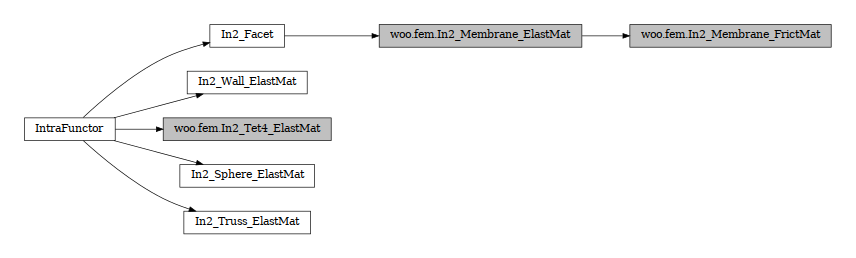class woo.dem.IntraFunctor(*args, **kwargs)

Functor appying internal forces

1. __init__(self: woo.dem.IntraFunctor) -> None

2. __init__(self: woo.dem.IntraFunctor, *args, **kwargs) -> None

#### In2_Sphere_ElastMat¶

class woo.dem.In2_Sphere_ElastMat(*args, **kwargs)

Apply contact forces on sphere; having one node only, Sphere generates no internal forces as such.

1. __init__(self: woo.dem.In2_Sphere_ElastMat) -> None

2. __init__(self: woo.dem.In2_Sphere_ElastMat, *args, **kwargs) -> None

alreadyWarned_ContactLoopWithApplyForces(= False)

Keep track of whether the warning on ContactLoop already applying forces was issued.

[type: bool, not shown in the UI]

#### In2_Wall_ElastMat¶

class woo.dem.In2_Wall_ElastMat(*args, **kwargs)

Apply contact forces on wall. Wall generates no internal forces as such. Torque from applied forces is discarded, as Wall does not rotate.

1. __init__(self: woo.dem.In2_Wall_ElastMat) -> None

2. __init__(self: woo.dem.In2_Wall_ElastMat, *args, **kwargs) -> None

#### In2_Truss_ElastMat¶

class woo.dem.In2_Truss_ElastMat(*args, **kwargs)

Compute elastic response of cylinder determined by 2 nodes.

1. __init__(self: woo.dem.In2_Truss_ElastMat) -> None

2. __init__(self: woo.dem.In2_Truss_ElastMat, *args, **kwargs) -> None

setL0(= True)

Automatically set equilibrium length of truss, when first encountered.

[type: bool]

#### In2_Facet¶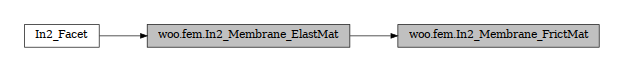class woo.dem.In2_Facet(*args, **kwargs)

Distribute frce on Facet to its nodes; the algorithm is purely geometrical since Facet has no internal forces, therefore can accept any kind of material.

1. __init__(self: woo.dem.In2_Facet) -> None

2. __init__(self: woo.dem.In2_Facet, *args, **kwargs) -> None

## AnisoPorosityAnalyzer¶

class woo.dem.AnisoPorosityAnalyzer(*args, **kwargs)

Engine which analyzes current scene and computes directionaly porosity value by intersecting spheres with lines. The algorithm only works on periodic simulations.

1. __init__(self: woo.dem.AnisoPorosityAnalyzer) -> None

2. __init__(self: woo.dem.AnisoPorosityAnalyzer, *args, **kwargs) -> None

poro(= Matrix3(0, 0, 0, 0, 0, 0, 0, 0, 0))

Store analysis result here

div(= 10)

Fineness of division of interval (0…1) for $$u$$,$$v$$ ∈〈0…1〉, which are used for uniform distribution over the positive octant as $$\theta= rac{\pi}{2}u$$, $$\phi=\arccos v$$ (see http://mathworld.wolfram.com/SpherePointPicking.html)

[type: int]

initStep(= -1)

Step in which internal data were last updated

[type: long, not accessible from python]

initNum(= 18446744073709551615)

Number of particles at last update

[type: size_t, not accessible from python]

rayIds(= [])

Particles intersected with ray when oneRay was last called from python.

rayPts(= [])

Starting and ending points of segments intersecting particles.

clearVis(self: woo.dem.AnisoPorosityAnalyzer)None

Clear visualizable intersection segments

oneRay(*args, **kwargs)

1. oneRay(self: woo.dem.AnisoPorosityAnalyzer, A: _wooEigen11.Vector3, B: _wooEigen11.Vector3 = Vector3(0,0,0), vis: bool = True) -> float

2. oneRay(self: woo.dem.AnisoPorosityAnalyzer, theta: float, phi: float, vis: bool = True) -> float

relSolid(self: woo.dem.AnisoPorosityAnalyzer, theta: float, phi: float, pt0: _wooEigen11.Vector3 = Vector3(0, 0, 0), vis: bool = False)float
static splitRay(theta: float, phi: float, pt0: _wooEigen11.Vector3 = Vector3(0, 0, 0), T: _wooEigen11.Matrix3 = Matrix3(1, 0, 0, 0, 1, 0, 0, 0, 1))List[_wooEigen11.Vector3]

## Suspicious¶

class woo.dem.Suspicious(*args, **kwargs)

Watch the simulation and signal suspicious evolutions, such as sudden increase in contact force beyond usual measure or unsual velocity.

1. __init__(self: woo.dem.Suspicious) -> None

2. __init__(self: woo.dem.Suspicious, *args, **kwargs) -> None

avgVel(= nan)

Average velocity norm.

avgForce(= nan)

Average particle force norm.

avgFn(= nan)

Average normal force norm.

avgFt(= nan)

Average shear force norm.

avgUn(= nan)

Average normal overlap.

relThreshold(= 100.0)

Break on quantity this much larger than the average.

[type: Real]

errPar(= _8ParticleList[])

Particle where there was some error (shown in OpenGL).

[type: vector<shared_ptr<Particle>>]

errCon(= [])

Contacts where there was some error (shown in OpenGL)

[type: vector<shared_ptr<Contact>>]

## ForcesToHdf5¶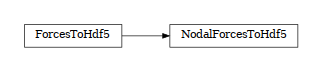class woo.dem.ForcesToHdf5(*args, **kwargs)

Periodically export nodal/contact forces to HDF5 file. Nodal forces are only exported for tagged nodes (having DemDataTagged attached, instead of plain DemData) and include both force and torque. Contact forces only export force (not torque, which is zero for most models, but the adaptation would be easy).

1. __init__(self: woo.dem.ForcesToHdf5) -> None

2. __init__(self: woo.dem.ForcesToHdf5, *args, **kwargs) -> None

what(= 0)

Select whether to export nodal forces (default) or contact forces.

[type: int, named enum, possible values are: ‘node’ (‘nodes’, ‘nodal’; 0), ‘contact’ (‘contacts’; 1)]

contMask(= 2)

Only export contacts where at least one particle matches this mask. If zero, match all contacts. If this Contact.pA has matching mask, inverts force sense; this has as result that (unless both particles match) the force is exported as it acts on the matching particle.

[type: int]

out(= '')

Name of the output file.

[type: string]

deflate(= 9)

Compression level for HDF5 chunked storage; valid values are 0 to 9 (will be clamped if outside).

[type: int]

node(= None)

Local coordinate system; if defined, contact forces, torques and positions will be fransfored to local coordinates.

[type: shared_ptr<Node>]

### NodalForcesToHdf5¶

class woo.dem.NodalForcesToHdf5(*args, **kwargs)

Legacy class which only serves for backwards compatibility.

1. __init__(self: woo.dem.NodalForcesToHdf5) -> None

2. __init__(self: woo.dem.NodalForcesToHdf5, *args, **kwargs) -> None

## Collision detection¶

TODO

### Collider¶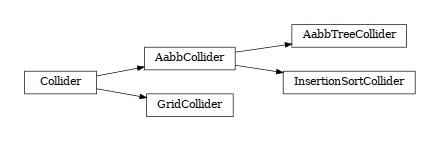class woo.dem.Collider(*args, **kwargs)

Abstract class for finding spatial collisions between bodies.

1. __init__(self: woo.dem.Collider) -> None

2. __init__(self: woo.dem.Collider, *args, **kwargs) -> None

nFullRuns(= 0)

Cumulative number of full runs, when collision detection is needed.

[type: int]

static mayCollide(dem: woo.dem.DemField, pA: woo.dem.Particle, pB: woo.dem.Particle)bool

Predicate whether two particles in question may collide or not

probeAabb(self: woo.dem.Collider, mn: _wooEigen11.Vector3, mx: _wooEigen11.Vector3)List[int]

Return list of particles intersected by axis-aligned box with given corners

### AabbCollider¶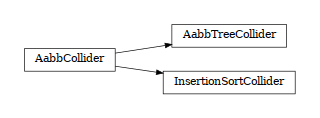class woo.dem.AabbCollider(*args, **kwargs)

Abstract class for colliders based on axis-aligned bounding boxes.

1. __init__(self: woo.dem.AabbCollider) -> None

2. __init__(self: woo.dem.AabbCollider, *args, **kwargs) -> None

boundDispatcher(= <BoundDispatcher @ 0x15bec60>)

BoundDispatcher object that is used for creating bounds on collider’s request as necessary.

[type: shared_ptr<BoundDispatcher>, read-only in python]

verletDist(= -0.05)

Length by which to enlarge particle bounds, to avoid running collider at every step. Stride disabled if zero, and bounding boxes are updated at every step. Negative value will trigger automatic computation, so that the real value will be |verletDist| × minimum spherical particle radius and minimum Inlet radius (for particles which don’t exist yet); if there is no minimum radius found, it will be set to 0.0 (with a warning) and disabled.

[type: Real]

noBoundOk(= False)

Allow particles without bounding box. This is currently only useful for testing woo.fem.Tetra which don’t undergo any collisions.

[type: bool]

#### AabbTreeCollider¶

class woo.dem.AabbTreeCollider(*args, **kwargs)

Collider based on AABB hierarchy.

1. __init__(self: woo.dem.AabbTreeCollider) -> None

2. __init__(self: woo.dem.AabbTreeCollider, *args, **kwargs) -> None

#### InsertionSortCollider¶

class woo.dem.InsertionSortCollider(*args, **kwargs)

Collider with O(n log(n)) complexity, using Aabb for bounds.

At the initial step, Bodies’ bounds (along sortAxis) are first std::sort’ed along one axis (sortAxis), then collided. The initial sort has $$O(n^2)$$ complexity, see Colliders’ performance for some information (There are scripts in examples/collider-perf for measurements).

Insertion sort is used for sorting the bound list that is already pre-sorted from last iteration, where each inversion calls checkOverlap which then handles either overlap (by creating interaction if necessary) or its absence (by deleting interaction if it is only potential).

Bodies without bounding volume (such as clumps) are handled gracefully and never collide. Deleted bodies are handled gracefully as well.

This collider handles periodic boundary conditions. There are some limitations, notably:

1. No body can have Aabb larger than cell’s half size in that respective dimension. You get exception it it does and gets in interaction.

2. No body can travel more than cell’s distance in one step; this would mean that the simulation is numerically exploding, and it is only detected in some cases.

Stride can be used to avoid running collider at every step by enlarging the particle’s bounds, tracking their velocities and only re-run if they might have gone out of that bounds (see Verlet list for brief description and background) . This requires cooperation from Leapfrog as well as BoundDispatcher, which will be found among engines automatically (exception is thrown if they are not found).

If you wish to use strides, set verletDist (length by which bounds will be enlarged in all directions) to some value, e.g. 0.05 × typical particle radius. This parameter expresses the tradeoff between many potential interactions (running collider rarely, but with longer exact interaction resolution phase) and few potential interactions (running collider more frequently, but with less exact resolutions of interactions); it depends mainly on packing density and particle radius distribution.

1. __init__(self: woo.dem.InsertionSortCollider) -> None

2. __init__(self: woo.dem.InsertionSortCollider, *args, **kwargs) -> None

forceInitSort(= False)

When set to true, full sort will be run regardless of other conditions. This flag is then reset automatically to false

[type: bool]

sortAxis(= 0)

Axis for the initial contact detection.

[type: int]

sortThenCollide(= False)

Separate sorting and colliding phase; it is MUCH slower, but all interactions are processed at every step; this effectively makes the collider non-persistent, not remembering last state. (The default behavior relies on the fact that inversions during insertion sort are overlaps of bounding boxes that just started/ceased to exist, and only processes those; this makes the collider much more efficient.)

[type: bool]

maxVel2(= 0.0)

Maximum encountered velocity of a particle, to compute bounding box shift.

numReinit(= 0)

Cumulative number of bound array re-initialization.

stepInvs(= Vector3i(0, 0, 0))

Number of inversions in insertion sort in the last step; always zero in the non-debug builds

[type: Vector3i]

numInvs(= Vector3i(0, 0, 0))

Cumulative number of inversions in insertion sort; always zero in the non-debug builds

[type: Vector3i]

ompTuneSort(= Vector3i(1, 1000, 0))

Fine-tuning for the OpenMP-parallellized partial insertion sort. The first number is the number of chunks per CPU (2 means each core will process 2 chunks sequentially, on average). The second number (if positive) is the lower bound on number of particles per chunk; the third number (if positive) is the limit of bounds per one chunk (15000 means that if there are e.g. 300k particles, bounds will be processed in 20 chunks, even if the number of chunks from the first number is smaller).

[type: Vector3i]

sortChunks(= -1)

Number of threads that were actually used during the last parallelized insertion sort.

paraPeri(= False)

(debugging only): enable/disable(default) parallel sort with periodic boundaries.

[type: bool]

periDbgNew(= False)

Compute periodic overlaps and periods twice (with the original and the new algorithm) compare the results and report discrepancies.

[type: bool]

maxSortPass(= -100)

If partial sort is not done after this many passes, give up. Usually more than a few passes (with non-parallelized insertion sort) already means a particle went crazy or the whole simulation is exploding. Negative value is relative to the number of cores as parallel insertion sort is done per chunks and more chunks mean more passes might be necessary.

[type: int]

periodic(= False)

Whether the collider is in periodic mode (read-only; for debugging)

[type: bool, not saved, read-only in python]

dbgInfo(self: woo.dem.InsertionSortCollider)object

Return python distionary with information on some internal structures (debugging only)

dumpBounds(self: woo.dem.InsertionSortCollider)tuple

Return representation of the internal sort data. The format is ([...],[...],[...]) for 3 axes, where each ... is a list of entries (bounds). The entry is a tuple with the fllowing items:

• coordinate (float)

• body id (int), but negated for negative bounds

• period numer (int), if the collider is in the periodic regime.

property maxima

Array of maximum bbox coords; every 3 contiguous values are x, y, z for one particle

property minima

Array of minimum bbox coords; every 3 contiguous values are x, y,z for one particle

spatialOverlap(self: woo.dem.InsertionSortCollider, scene: woo.core.Scene, id1: int, id2: int)object

### GridCollider¶

class woo.dem.GridCollider(*args, **kwargs)

Grid-based collider.

1. __init__(self: woo.dem.GridCollider) -> None

2. __init__(self: woo.dem.GridCollider, *args, **kwargs) -> None

► Grid geometry

domain(= AlignedBox3((0, 0, 0), (1, 1, 1)))

Domain spanned by the grid.

[type: AlignedBox3r]

minCellSize(= 1.0)

Minimum cell size which will be used to compute dim.

[type: Real]

dim(= Vector3i(-1, -1, -1))

Number of cells along each axis

cellSize(= Vector3(nan, nan, nan))

Actual cell size

► Data

gridPrev(= None)

Previous fully populated grid.

[type: shared_ptr<GridStore>, not saved]

gridCurr(= None)

Current fully populated grid.

[type: shared_ptr<GridStore>, not saved]

gridOld(= None)

Grid containing entries in gridPrev but not in gridCurr.

[type: shared_ptr<GridStore>, not saved]

gridNew(= None)

Grid containing entries in gridCurr but not in gridPrev.

[type: shared_ptr<GridStore>, not saved]

► Rendering

color(= Vector3(1, 1, 0))

Color for rendering the domain

[type: Vector3r]

renderCells(= False)

Render cells.

[type: bool]

minOccup(= 0)

Minimum occupancy for cell to be rendered (zero cells are never rendered).

[type: int]

occupancyRange(= None)

Range for coloring grids based on occupancy (automatically created)

[type: shared_ptr<ScalarRange>]

► Tunables

gridDense(= 6)

Length of dense storage for new GridStore objects.

[type: int]

exIniSize(= 6)

GridStore.exIniSize for new grids.

[type: int]

exNumMaps(= 100)

GridStore.exNumMaps for new grids.

[type: int]

verletDist(= 0.0)

Length by which particle size is enalrged, to avoid running the collider at every timestep.

[type: Real, unit: m]

verletSteps(= 0)

If positive, enlarge boxes of some particle nodes (currently only spheres are supported) so that they will still be inside the box after verletSteps with their current velocity; verletDist is still used when velocity is too small.

[type: int]

complSetMinSize(= -1)

The value of setMinSize when calling GridStore.computeRelativeComplements.

[type: int]

useDiff(= True)

Create new contacts based on set complement of gridPrev with respect to gridCurr if both contain meaningful data and are compatible; if false, always traverse gridCurr and try adding all possible contacts (this should be much slower)

[type: bool]

around(= False)

If frue, particle in every cell is checked with particles in all cells around; this makes the grid storage substantially less loaded, as all particles can be shrunk by one half of the cell size.

Warning

this feature is broken and will raise exception if enabled; the trade-off is not good, since many more cells need to be checked around every cell, and many more potential contacts are created.

[type: bool]

shrink(= 0.0)

The amount of shrinking for each particle (half of the minimum cell size if around is true, zero otherwise.

[type: Real, not shown in the UI, read-only in python]

boundDispatcher(= <GridBoundDispatcher @ 0x1611110>)

Dispatches GridBound creation to GridBoundFuctor based on Shape type.

[type: shared_ptr<GridBoundDispatcher>]

#### GridStore¶

class woo.dem.GridStore(*args, **kwargs)

3d grid storing scalar (particles ids) in partially dense array; the grid is actually 4d (gridSize×cellLen), and each cell may contain additional items in separate mapped storage, if the cellLen is not big enough to accomodate required number of items. Appending to cells is guarded via mutexes (with denseLock) optionally, appending to extra storage is mutex-protected always. Write acces from python should be used for testing exclusively.

1. __init__(self: woo.dem.GridStore) -> None

2. __init__(self: woo.dem.GridStore, *args, **kwargs) -> None

gridSize(= Vector3i(1, 1, 1))

Dimension of the grid.

cellLen(= 4)

Size of the dense storage in each cell

denseLock(= True)

Whether this grid supports per-cell dense storage locking for appending (must use protected_append)

exIniSize(= 4)

Initial size of extension vectors, and step of their growth if needed.

exNumMaps(= 10)

Number of maps for extra items not fitting the dense storage (it affects how fine-grained is locking for those extra elements)

lo(= Vector3(nan, nan, nan))

Lower corner of the domain.

[type: Vector3r]

cellSize(= Vector3(nan, nan, nan))

Spatial size of the grid cell.

[type: Vector3r]

__delitem__(self: woo.dem.GridStore, arg0: _wooEigen11.Vector3i)None
__getitem__(self: woo.dem.GridStore, arg0: _wooEigen11.Vector3i)list
__setitem__(self: woo.dem.GridStore, arg0: _wooEigen11.Vector3i, arg1: List[int])None
append(self: woo.dem.GridStore, ijk: _wooEigen11.Vector3i, id: int)None

Append new element; uses mutexes with denseLock

complements(self: woo.dem.GridStore, B: woo.dem.GridStore, setMinSize: int = - 1)tuple
countEx(self: woo.dem.GridStore)dict

Return dictionary mapping ijk to number of items in the extra storage.

counts(self: woo.dem.GridStore)List[int]

Return array with number of cells with given number of elements: [number of cells with 0 elements, number of cells with 1 elements, …]

ijk2box(self: woo.dem.GridStore, ijk: _wooEigen11.Vector3i)_wooEigen11.AlignedBox3

Box for given cell

ijk2lin(self: woo.dem.GridStore, arg0: _wooEigen11.Vector3i)int
lin2ijk(self: woo.dem.GridStore, arg0: int)_wooEigen11.Vector3i
size(self: woo.dem.GridStore, arg0: _wooEigen11.Vector3i)int
xyz2ijk(self: woo.dem.GridStore, arg0: _wooEigen11.Vector3)_wooEigen11.Vector3i

Convert spatial coordinates to cell coordinate (no bound checking is done)

xyzNearIjk(self: woo.dem.GridStore, from: _wooEigen11.Vector3i, ijk: _wooEigen11.Vector3i)_wooEigen11.Vector3

Return point nearest to from (given in cell coords) in cell ijk

xyzNearXyz(self: woo.dem.GridStore, from: _wooEigen11.Vector3, ijk: _wooEigen11.Vector3i)_wooEigen11.Vector3

Return point nearest to from*(given in spatial coords) in cell *ijk (corner, at a face, on an edge, inside)

### Bound¶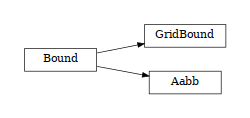class woo.dem.Bound(*args, **kwargs)

Object bounding the associated body.

1. __init__(self: woo.dem.Bound) -> None

2. __init__(self: woo.dem.Bound, *args, **kwargs) -> None

box(= AlignedBox3((1.7976931348623157e308, 1.7976931348623157e308, 1.7976931348623157e308), (-1.7976931348623157e308, -1.7976931348623157e308, -1.7976931348623157e308)))

Axis-aligned bounding box.

[type: AlignedBox3r, unit: m, not saved, read-only in python]

dispHierarchy(self: woo.dem.Bound, names: bool = True)list

Return list of dispatch classes (from down upwards), starting with the class instance itself, top-level indexable at last. If names is true (default), return class names rather than numerical indices.

property dispIndex

Return class index of this instance.

#### GridBound¶

class woo.dem.GridBound(*args, **kwargs)

Bound defined via grid cell indices (used with GridCollider)

1. __init__(self: woo.dem.GridBound) -> None

2. __init__(self: woo.dem.GridBound, *args, **kwargs) -> None

nodePlay(= [])

Space in which respective nodes of the shapes may be without triggering new contact detection

#### Aabb¶

class woo.dem.Aabb(*args, **kwargs)

Axis-aligned bounding box, for use with InsertionSortCollider.

1. __init__(self: woo.dem.Aabb) -> None

2. __init__(self: woo.dem.Aabb, *args, **kwargs) -> None

nodeLastPos(= [])

Node positions when bbox was last updated.

[type: vector<Vector3r>, unit: m, read-only in python]

maxD2(= 0.0)

Maximum allowed squared distance for nodal displacements (i.e. how much was the bbox enlarged last time)

[type: Real, unit: m², not shown in the UI, read-only in python]

maxRot(= nan)

Maximum allowed rotation (in radians, without discriminating different angles) that does not yet invalidate the bbox. Functor sets to -1 (or other negative value) for particles where node rotation does not influence the box (such as spheres or facets); in that case, orientation difference is not computed at all. If it is left at NaN, it is an indication that the functor does not implemnt this behavior and an error will be raised in the collider.

nodeLastOri(= [])

Node orientations when bbox was last updated.

### BoundFunctor¶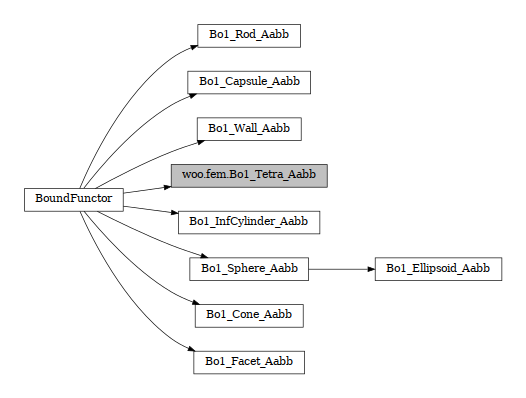class woo.dem.BoundFunctor(*args, **kwargs)

Functor for creating/updating woo.dem.Bound.

1. __init__(self: woo.dem.BoundFunctor) -> None

2. __init__(self: woo.dem.BoundFunctor, *args, **kwargs) -> None

#### Bo1_Rod_Aabb¶

class woo.dem.Bo1_Rod_Aabb(*args, **kwargs)

Compute woo.dem.Aabb of a Rod particle

1. __init__(self: woo.dem.Bo1_Rod_Aabb) -> None

2. __init__(self: woo.dem.Bo1_Rod_Aabb, *args, **kwargs) -> None

#### Bo1_Facet_Aabb¶

class woo.dem.Bo1_Facet_Aabb(*args, **kwargs)

Creates/updates an Aabb of a Facet.

1. __init__(self: woo.dem.Bo1_Facet_Aabb) -> None

2. __init__(self: woo.dem.Bo1_Facet_Aabb, *args, **kwargs) -> None

#### Bo1_InfCylinder_Aabb¶

class woo.dem.Bo1_InfCylinder_Aabb(*args, **kwargs)

Creates/updates an Aabb of a InfCylinder

1. __init__(self: woo.dem.Bo1_InfCylinder_Aabb) -> None

2. __init__(self: woo.dem.Bo1_InfCylinder_Aabb, *args, **kwargs) -> None

#### Bo1_Sphere_Aabb¶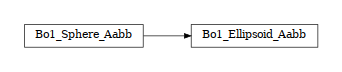class woo.dem.Bo1_Sphere_Aabb(*args, **kwargs)

Functor creating Aabb from Sphere. Honors DemField.distFactor.

1. __init__(self: woo.dem.Bo1_Sphere_Aabb) -> None

2. __init__(self: woo.dem.Bo1_Sphere_Aabb, *args, **kwargs) -> None

distFactor(= -1.0)

removed in API 10103, set DemField.distFactor instead.

[type: Real, not shown in the UI, not dumped, DEPRECATED, raises ValueError when accessed]

##### Bo1_Ellipsoid_Aabb¶
class woo.dem.Bo1_Ellipsoid_Aabb(*args, **kwargs)

Functor creating Aabb from Ellipsoid.

Todo

Handle rotation which is not detected by verlet distance!

1. __init__(self: woo.dem.Bo1_Ellipsoid_Aabb) -> None

2. __init__(self: woo.dem.Bo1_Ellipsoid_Aabb, *args, **kwargs) -> None

#### Bo1_Capsule_Aabb¶

class woo.dem.Bo1_Capsule_Aabb(*args, **kwargs)

Creates/updates an Aabb of a Capsule

1. __init__(self: woo.dem.Bo1_Capsule_Aabb) -> None

2. __init__(self: woo.dem.Bo1_Capsule_Aabb, *args, **kwargs) -> None

#### Bo1_Cone_Aabb¶

class woo.dem.Bo1_Cone_Aabb(*args, **kwargs)

Compute woo.dem.Aabb of a Cone particle

1. __init__(self: woo.dem.Bo1_Cone_Aabb) -> None

2. __init__(self: woo.dem.Bo1_Cone_Aabb, *args, **kwargs) -> None

#### Bo1_Wall_Aabb¶

class woo.dem.Bo1_Wall_Aabb(*args, **kwargs)

Creates/updates an Aabb of a Wall

1. __init__(self: woo.dem.Bo1_Wall_Aabb) -> None

2. __init__(self: woo.dem.Bo1_Wall_Aabb, *args, **kwargs) -> None

### GridBoundFunctor¶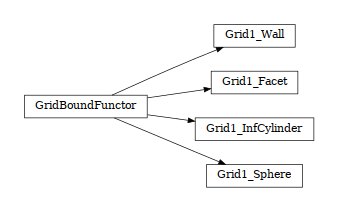class woo.dem.GridBoundFunctor(*args, **kwargs)

Functor for creating/updating woo.dem.GridBound.

1. __init__(self: woo.dem.GridBoundFunctor) -> None

2. __init__(self: woo.dem.GridBoundFunctor, *args, **kwargs) -> None

#### Grid1_Sphere¶

class woo.dem.Grid1_Sphere(*args, **kwargs)

Functor filling GridStore from Sphere, used with GridCollider.

1. __init__(self: woo.dem.Grid1_Sphere) -> None

2. __init__(self: woo.dem.Grid1_Sphere, *args, **kwargs) -> None

distFactor(= -1.0)

removed in API 10103, set DemField.distFactor instead.

[type: Real, not shown in the UI, not dumped, DEPRECATED, raises ValueError when accessed]

#### Grid1_Facet¶

class woo.dem.Grid1_Facet(*args, **kwargs)

Functor filling GridStore from Facet, used with GridCollider.

1. __init__(self: woo.dem.Grid1_Facet) -> None

2. __init__(self: woo.dem.Grid1_Facet, *args, **kwargs) -> None

movable(= False)

Set to allow movable facets (with grid enlarged by GridCollider.verletDist. If false and a moving facet is encountered, an exception is raised.

[type: bool]

#### Grid1_InfCylinder¶

class woo.dem.Grid1_InfCylinder(*args, **kwargs)

Functor filling GridStore from InfCylinder, used with GridCollider.

1. __init__(self: woo.dem.Grid1_InfCylinder) -> None

2. __init__(self: woo.dem.Grid1_InfCylinder, *args, **kwargs) -> None

movable(= False)

Set to allow movable cylinders (with grid enlarged by GridCollider.verletDist. If false and a moving cylinder is encountered, an exception is raised.

[type: bool]

#### Grid1_Wall¶

class woo.dem.Grid1_Wall(*args, **kwargs)

Functor filling GridStore from Wall, used with GridCollider.

1. __init__(self: woo.dem.Grid1_Wall) -> None

2. __init__(self: woo.dem.Grid1_Wall, *args, **kwargs) -> None

movable(= False)

Set to allow movable walls (with grid enlarged by GridCollider.verletDist. If false and a movable wall is encountered, an exception is raised.

[type: bool]

### BoundDispatcher¶

class woo.dem.BoundDispatcher(*args, **kwargs)

Dispatcher calling functors based on received argument type(s).

1. __init__(self: woo.dem.BoundDispatcher) -> None

2. __init__(self: woo.dem.BoundDispatcher, *args, **kwargs) -> None

functors(= [])

Functors active in the dispatch mechanism [overridden below].

[type: vector<shared_ptr<BoundFunctor>>]

dispFunctor(self: woo.dem.BoundDispatcher, arg0: woo.dem.Shape)woo.dem.BoundFunctor

Return functor that would be dispatched for given argument(s); None if no dispatch; ambiguous dispatch throws.

dispMatrix(self: woo.dem.BoundDispatcher, names: bool = True)dict

Return dictionary with contents of the dispatch matrix.

### GridBoundDispatcher¶

class woo.dem.GridBoundDispatcher(*args, **kwargs)

Dispatcher calling functors based on received argument type(s).

1. __init__(self: woo.dem.GridBoundDispatcher) -> None

2. __init__(self: woo.dem.GridBoundDispatcher, *args, **kwargs) -> None

functors(= [])

Functors active in the dispatch mechanism [overridden below].

[type: vector<shared_ptr<GridBoundFunctor>>]

dispFunctor(self: woo.dem.GridBoundDispatcher, arg0: woo.dem.Shape)woo.dem.GridBoundFunctor

Return functor that would be dispatched for given argument(s); None if no dispatch; ambiguous dispatch throws.

dispMatrix(self: woo.dem.GridBoundDispatcher, names: bool = True)dict

Return dictionary with contents of the dispatch matrix.

## Packings¶

TODO

### ShapePack¶

class woo.dem.ShapePack(*args, **kwargs)

Representation of geometry of many particles, with the ability of text I/O. It is meant as a replacement for woo.pack.SpherePack, which only handles spherical particles.

1. __init__(self: woo.dem.ShapePack) -> None

2. __init__(self: woo.dem.ShapePack, *args, **kwargs) -> None

cellSize(= Vector3(0, 0, 0))

Positive components signify periodic boundary along the respective axis.

[type: Vector3r]

movable(= False)

Whether the packing is movable, i.e. should be automatically recentered after filtered with a predicate.

[type: bool]

div(= 5)

Default value for recomputing properties of clumps (relative to the smallest equivalent radius)

[type: int]

raws(= [])

Raw shapes of particles/clumps.

[type: vector<shared_ptr<ShapeClump>>]

userData(= '')

String of arbitrary user data to be loaded/saved with the ShapePack.

[type: string]

loadFrom(= '')

If given when constructing the instance, the file is loaded immediately and the attribute is reset.

[type: string]

add(self: woo.dem.ShapePack, shapes: List[woo.dem.Shape], clumped: bool = True)None
canonicalize(self: woo.dem.ShapePack)None
cellRepeat(self: woo.dem.ShapePack, arg0: _wooEigen11.Vector3i)None
filter(self: woo.dem.ShapePack, predicate: Predicate, recenter: int = - 1)None
filtered(self: woo.dem.ShapePack, predicate: Predicate, recenter: int = - 1)woo.dem.ShapePack
fromDem(self: woo.dem.ShapePack, scene: woo.core.Scene, dem: woo.dem.DemField, mask: int = 0, skipUnsupported: bool = True)None
load(self: woo.dem.ShapePack, arg0: str)None
loadTxt(self: woo.dem.ShapePack, arg0: str)None
save(self: woo.dem.ShapePack, arg0: str)None
saveTxt(self: woo.dem.ShapePack, arg0: str)None
solidVolume(self: woo.dem.ShapePack)float
toDem(self: woo.dem.ShapePack, scene: woo.core.Scene, dem: woo.dem.DemField, mat: woo.dem.Material, mask: int = 5, color: float = nan)None
translate(self: woo.dem.ShapePack, arg0: _wooEigen11.Vector3)None

### RawShape¶

class woo.dem.RawShape(*args, **kwargs)

Object holding raw geometry data for one Shape in a uniform manner: center and radius of bounding sphere, plus an array of raw data. It is used as an intermediary shape-neutral storage format.

1. __init__(self: woo.dem.RawShape) -> None

2. __init__(self: woo.dem.RawShape, *args, **kwargs) -> None

className(= '')

Name of the Shape subclass.

[type: string]

center(= Vector3(0, 0, 0))

Center of the bounding sphere.

[type: Vector3r]

radius(= 0.0)

[type: Real]

raw(= [])

Raw data for shape reconstruction; the size of the array is shape-specific.

[type: vector<Real>]

### ShapeClump¶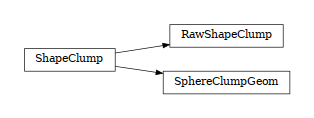class woo.dem.ShapeClump(*args, **kwargs)

Defines pure geometry of clumps. This is a base class, not to be used as-is.

1. __init__(self: woo.dem.ShapeClump) -> None

2. __init__(self: woo.dem.ShapeClump, *args, **kwargs) -> None

scaleProb(= [])

Used by particle generators: piecewise-linear function probability(equivRad) given as a sequence of x,y coordinates. If not given, constant function $$p(d)=1$$ is assumed. See the documentation of woo.dem.PsdClumpGenerator for details.

[type: vector<Vector2r>]

pos(= Vector3(0, 0, 0))

Centroid position (computed automatically)

[type: Vector3r, not dumped, read-only in python]

ori(= Quaternion((1, 0, 0), 0))

Principal axes orientation (computed automatically)

[type: Quaternionr, not dumped, read-only in python]

volume(= nan)

Volume (computed automatically)

[type: Real, unit: m³, not dumped, read-only in python]

equivRad(= nan)

Equivalent radius of the clump (computed automatically) – mean of radii of gyration, i.e. $$\frac{1}{3}\sum \sqrt{I_{ii}/V}$$.

[type: Real, unit: m, not dumped, read-only in python]

inertia(= Vector3(nan, nan, nan))

Geometrical inertia (computed with unit density)

[type: Vector3r, not dumped, read-only in python]

div(= 5)

Sampling grid fineness, when computing volume and other properties, relative to the smallest sphere’s radius. When zero or negative, assume spheres don’t intersect and use a different algorithm (Steiner’s theorem).

[type: int, not dumped]

clumped(= True)

Whether nodes of this shape are clumped together when the particle is created (so far, clumped shapes only are produced).

makeParticles(self: woo.dem.ShapeClump, mat: woo.dem.Material, pos: _wooEigen11.Vector3, ori: _wooEigen11.Quaternion = Quaternion((1, 0, 0), 0), scale: float = 1.0, mask: int = 5)tuple

Create particle(s) as described by this geometry, positioned in pos and rotated with ori. Geometry will be scaled by scale. Returns tuple ([Node,…],[Particle,..]).

recompute(self: woo.dem.ShapeClump, div: int = 5, failOk: bool = False, fastOnly: bool = False)None

Recompute principal axes of the clump, using div for subdivision (see div for the semantics). failOk (silently return in case of invalid data) and fastOnly (return if there is lots of cells in subdivision) are only to be used internally.

#### SphereClumpGeom¶

class woo.dem.SphereClumpGeom(*args, **kwargs)

Defines geometry of spherical clumps. Each clump is described by spheres it is made of (position and radius).

1. __init__(self: woo.dem.SphereClumpGeom) -> None

2. __init__(self: woo.dem.SphereClumpGeom, *args, **kwargs) -> None

centers(= [])

Centers of constituent spheres, in clump-local coordinates.

[type: vector<Vector3r>]

radii(= [])

[type: vector<Real>]

static fromSpherePack(pack: SpherePack, div: int = 5)List[woo.dem.SphereClumpGeom]

Return [ SphereClumpGeom ] which contain all clumps and spheres from given SpherePack.

#### RawShapeClump¶

class woo.dem.RawShapeClump(*args, **kwargs)

Clump consisting of generic shapes (RawShape).

1. __init__(self: woo.dem.RawShapeClump) -> None

2. __init__(self: woo.dem.RawShapeClump, *args, **kwargs) -> None

rawShapes(= [])

Data for creating primitive shapes

[type: vector<shared_ptr<RawShape>>]

## Export¶

TODO

### VtkExport¶

class woo.dem.VtkExport(*args, **kwargs)

Export DEM simulation to VTK files for post-processing.

1. __init__(self: woo.dem.VtkExport) -> None

2. __init__(self: woo.dem.VtkExport, *args, **kwargs) -> None

out(= '')

Filename prefix to write into; woo.core.Scene.tags written as {tagName} are expanded at the first run.

[type: string]

compress(= True)

Compress output XML files

[type: bool]

ascii(= False)

Store data as readable text in the XML file (sets vtkXMLWriter data mode to vtkXMLWriter::Ascii, while the default is Appended

[type: bool]

multiblock(= False)

Write to multi-block VTK files, rather than separate files; currently borken, do not use.

[type: bool]

mask(= 0)

If non-zero, only particles matching the mask will be exported.

[type: int]

what(= 47)

Select data to be saved (e.g. VtkExport.spheres|VtkExport.mesh, or use VtkExport.all for everything)

[type: int]

sphereSphereOnly(= False)

Only export contacts between two spheres (not sphere+facet and such)

[type: bool]

infError(= True)

Raise exception for infinite objects which don’t have the glAB attribute set properly.

[type: bool]

skipInvisible(= True)

Skip invisible particles

[type: bool]

savePos(= False)

Save positions of spheres (redundant information, but useful for coloring by position in Paraview.

[type: bool]

clip(= AlignedBox3((1.7976931348623157e308, 1.7976931348623157e308, 1.7976931348623157e308), (-1.7976931348623157e308, -1.7976931348623157e308, -1.7976931348623157e308)))

Only export particles of which first node is in the clip box (if given).

[type: AlignedBox3r]

staticMeshBit(= 8)

Bit for identifying static mesh particles (Facet, Wall, InfCylinder only) which will be exported only once.

[type: int]

staticMeshDone(= False)

Whether static mesh was already exported

[type: bool]

subdiv(= 16)

Subdivision fineness for circular objects (such as cylinders).

Note

Facets are rendered without rounded edges (they are closed flat).

Note

Ellipsoids triangulation is controlled via the ellLev parameter.

[type: int]

ellLev(= 0)

Tesselation level for exporting ellipsoids (0 = icosahedron, each level subdivides one triangle into three.

[type: int, range: 0−3]

thickFacetDiv(= 1)

Subdivision for woo.dem.Facet objects with non-zero woo.dem.Facet.halfThick; the value of -1 will use subdiv; 0 will render only faces, without edges; 1 will close the edge flat; higher values mean the number of subdivisions.

[type: int]

cylCaps(= True)

Render caps of InfCylinder (at InfCylinder.glAB).

[type: bool]

rodSurf(= False)

Export rods (and derived classes) as capsule-shaped triangulated surfaces; without this option, rods are exported as plain connecting lines.

[type: bool]

nanValue(= 0.0)

Use this number instead of NaN in entries, since VTK cannot read NaNs properly

[type: Real]

outFiles(= {})

Files which have been written out, keyed by what they contain: ‘spheres’,’mesh’,’con’.

[type: map_string_vector_string, not shown in the UI, read-only in python]

outTimes(= [])

Times at which files were written.

[type: vector<Real>, not shown in the UI, read-only in python]

outSteps(= [])

Steps at which files were written.

[type: vector<int>, not shown in the UI, read-only in python]

mkDir(= False)

Attempt to create directory for output files, if not present.

[type: bool]

prevCellNum(= Vector3i(0, 0, 0))

Previous cell array sized, for pre-allocation.

[type: Vector3i, not shown in the UI, not saved, read-only in python]

makePvdFiles(self: woo.dem.VtkExport)dict

Write PVD files (one file for each category) and return dictionary mapping category name to the PVD filename; this requires that all active categories were saved at each step. Time points are output in the PVD file.

### FlowAnalysis¶

class woo.dem.FlowAnalysis(*args, **kwargs)

Collect particle flow data in rectangular grid, watching different particle groups (radius ranges via dLim or groups by mask via masks – only one of them may be specified), and saving averages to as VTK uniform grid once finished.

Note

Only particles returning meaningful woo.dem.Shape.equivRad are considered, all other are ignored.

1. __init__(self: woo.dem.FlowAnalysis) -> None

2. __init__(self: woo.dem.FlowAnalysis, *args, **kwargs) -> None

box(= AlignedBox3((1.7976931348623157e308, 1.7976931348623157e308, 1.7976931348623157e308), (-1.7976931348623157e308, -1.7976931348623157e308, -1.7976931348623157e308)))

Domain in which the flow is to be analyzed; the box may glow slightly to accomodate integer number of cells. Do not change once there is some data alread. Do not change once there is some data already.

[type: AlignedBox3r]

node(= None)

Defines local coordinates system; it undefined, uses global coordinates.

[type: shared_ptr<Node>]

data

Grid data – 5d since each 3d point contains multiple entries, and there are multiple grids.

[type: boost_multi_array_real_5, not accessible from python]

dLim(= [])

Limiting diameter values, for defining fractions which are analyzed separately. Do not change when there is some data already.

[type: vector<Real>]

masks(= [])

Mask values for fractions; it is an error if a particle matches multiple masks. Do not change when there is some data already.

[type: vector<int>]

nFractions(= -1)

Number of fractions, defined via dLim or masks; set automatically.

cellSize(= nan)

Size of one cell in the box (in all directions); will be satisfied exactly at the expense of perhaps slightly growing box. Do not change once there is some data already.

[type: Real]

boxCells(= Vector3i(0, 0, 0))

Number of cells in the box (computed automatically)

mask(= 0)

Particles to consider in the flow analysis (0 to consider everything).

[type: int]

cellData(= False)

Write flow rate as cell data rather than point data.

[type: bool]

porosity(= False)

Compute (and export) solid ratio (1-porosity) for all spheroidal particles (requires triangulation of the domain at every step, hence slow and not enabled by default.

[type: bool]

matStateScalar(= -1)

If non-negative, index of material state to analyze.

[type: int]

matStateName(= '')

If given, used for VTK-export of the scalar; if not given, is filled form the first instance encountered automatically.

[type: string]

timeSpan(= 0.0)

Total time that the analysis has been running.

[type: Real]

color(= Vector3(1, 1, 0))

Color for rendering the domain

[type: Vector3r]

reset(self: woo.dem.FlowAnalysis)None

Reset all data so that next analysis will be run from virgin state.

vtkExport(self: woo.dem.FlowAnalysis, out: str)List[str]

Export all fractions separately, and also an overall flow (sum). out specifies prefix for all export filenames, the rest is created to describe the fraction or all for the sum. Exported file names are returned, the sum being at the very end. Internally calls vtkExportFractions for all fractions.

vtkExportFractions(self: woo.dem.FlowAnalysis, out: str, fractions: List[int])str

Export one single fraction to file named out. The extension .vti is added automatically. fractions specifies existing fraction numbers to export. If fractions are an empty list ([]), all fractions are exported at once.

vtkExportVectorOps(self: woo.dem.FlowAnalysis, out: str, fracA: List[int], fracB: List[int])str

Export operations on two fraction sets: cross-product, weighted differences and such, for segregation analysis. Appends .opt.vti to the output filename in out.

### POVRayExport¶

class woo.dem.POVRayExport(*args, **kwargs)

Export DEM simulation to POV-Ray input files (work in progress) for ray-tracing.

1. __init__(self: woo.dem.POVRayExport) -> None

2. __init__(self: woo.dem.POVRayExport, *args, **kwargs) -> None

out(= '')

Filename prefix to write into; woo.core.Scene.tags written as {tagName} are expanded at the first run.

[type: string]

mask(= 0)

If non-zero, only particles matching the mask will be exported.

[type: int]

skipInvisible(= True)

Skip invisible particles

[type: bool]

clip(= AlignedBox3((1.7976931348623157e308, 1.7976931348623157e308, 1.7976931348623157e308), (-1.7976931348623157e308, -1.7976931348623157e308, -1.7976931348623157e308)))

Only export particles of which first node is in the clip box (if given).

[type: AlignedBox3r]

frameCounter(= 0)

Counts exported frames and uses the number to name output files.

[type: int]

colorRange(= <ScalarRange @ 0x16e43d0>)

Range to map woo.dem.Shape.color to RGB for pigment specification.

[type: shared_ptr<ScalarRange>]

colorFuzz(= 0.2)

Relative fuzz for particle color; the texture is called with RGB colors mapped (via colorRange) from Shape.color - colorFuzz and Shape.color + colorFuzz, clamped to (0,1)).

[type: Real]

staticMask(= 8)

Mask for static particles, which will be exported only once into a special incude file separate from the per-frame include files.

[type: int]

masks(= [8, 4, 1])

Masks which will be tried one after another (first match counts) on each particle to determine which texture will be applied to it. Texture names are taken from textures and must be defined in the master file.

[type: vector<int>]

textures(= ['static', 'outlet', 'movable'])

Texture names applied to particles matching masks – the first matching mask counts, textures are named woo_tex_* and must be modified in the master file by the user (only an example definition is written by Woo). Particles not matching any mask will be assigned texture default (woo_tex_default). Each texture receives two rgb arguments which are colormapped from woo.dem.Shape.color using colorRange and colorFuzz.

[type: vector<string>]

cylCapTexture(= '')

If non-empty, InfCylinder objects will be drawn as several objects, with caps having this separate texture type.

[type: string]

wallTexture(= '')

If non-empty, Wall objects will have this texture applied, regardless of their mask. Meant for easily assigning checkerboard texture to wall objects.

[type: string]

texturesInc(= '')

Better textures, conditionally included in every step; if not provided, default filename will be generated.

[type: string]

extraInc(= ['woo-logo.inc'])

If set, these files will be conditionally included at the end of every step.

[type: vector<string>]

camLocation(= Vector3(15, 10, 0))

Default location of the camera

[type: Vector3r]

camLookAt(= Vector3(0, 0, 0))

Default look direction of the camera

[type: Vector3r]

camAngle(= 45.0)

Default camera angle (in degrees)

[type: float]

connMesh(= 1)

Whether to export facets (Facet and derived classes) as connected meshes, and in what cases. This entails building topology and determining connected components of the topology graph, which can demand non-negligible computation; the default is to do it for static mesh only, which is done only once, but not at every simulations steps. It is however necessary to set to always if non-static mesh is moving and should be exported.

Note

Facets are normally exported one-by-one. Mesh allows for more efficient processing in POV-Ray and for things like texture covering more facets.

[type: int, named enum, possible values are: ‘none’ (‘’, ‘never’; 0), ‘static’ (‘stat’, ‘static only’; 1), ‘always’ (‘yes’; 2)]

## Particle¶

Each particles in DEM is defined by its shape (given by multiple nodes) and other parameters.

### Particle¶

class woo.dem.Particle(*args, **kwargs)

Particle in DEM

1. __init__(self: woo.dem.Particle) -> None

2. __init__(self: woo.dem.Particle, *args, **kwargs) -> None

id(= -1)

Index in DemField::particles

mask(= 1)

Bitmask for collision detection and other (group 1 by default)

[type: uint]

shape(= None)

Geometrical configuration of the particle

[type: shared_ptr<Shape>]

material(= None)

Material of the particle

[type: shared_ptr<Material>]

matState(= None)

Material state of the particle (such as damage data and similar)

[type: shared_ptr<MatState>]

contacts(= {})

Contacts of this particle, indexed by id of the other particle.

[type: MapParticleContact, not saved, not accessible from python]

property Ek

Summary kinetic energy of the particle, shorthand for p.Ekt+p.Ekr.

property Ekr

Rotational kinetic energy of the particle, computed on-demand as $$\frac{1}{2}\vec{\omega}^T\mat{T}^T\mat{I}\mat{T}\omega$$ (where $$\mat{T}$$ is p.shape.nodes.ori as rotation matrix, $$\mat{I}$$ is p.shape.nodes.dem.inertia as diagonal matrix – uninodal particles only (raises exception otherwise). Space deformation is not considered here, see getEk.

property Ekt

Translational kinetic energy of the particle, computed on-demand as $$\frac{1}{2}m|\vec{v}|^2$$ – uninodal particles only (raises exception otherwise). Space deformation is not considered here, see getEk.

property allContacts

Return dictionary mapping other particles' IDs <Particle.id to Contact objects, including contacts which are not real.

property angVel

Particle angular velocity; shorthand for p.shape.nodes.dem.angVel – uninodal particles only (raises exception otherwise).

property blocked

Blocked degrees of freedom of the particle; shorthand for p.shape.nodes.dem.blocked – uninodal particles only (raises exception otherwise).

property con

Return list of IDs of contacting particles (real contacts only); shorthand for p.contacts.keys().

property f

Force on particle; shorthand for p.shape.nodes.dem.force – uninodal particles only (raises exception otherwise).

getEk(self: woo.dem.Particle, trans: bool = True, rot: bool = True, scene: woo.core.Scene = None)float

Compute kinetic energy (translational and/or rotational); when scene is given, only fluctuation linear/angular velocity will be considered if periodic boundary conditions are active.

property impose

Particle imposed constraints; shorthand for p.shape.nodes.dem.impose – uninodal particles only (raises exception otherwise).

property inertia

Particle inertia; shorthand for p.shape.nodes.dem.inertia – uninodal particles only (raises exception otherwise).

static make(shape: woo.dem.Shape, mat: woo.dem.Material, fixed: bool = False)woo.dem.Particle

Return Particle instance created from given shape and material; nodes with DemData are automatically added to the shape, mass and inertia is recomuted.

property mass

Particle mass; shorthand for p.shape.nodes.dem.mass – uninodal particles only (raises exception otherwise).

property mat

Material of the particle

property nodes

List of particle nodes; shorthand for p.shape.nodes.

property ori

Particle orientation; shorthand for p.shape.nodes.ori – uninodal particles only (raises exception otherwise).

property pos

Particle position; shorthand for p.shape.nodes.pos – uninodal particles only (raises exception otherwise).

property refPos

Reference particle position; shorthand for p.shape.nodes.gl.refPos, and Vector3(nan,nan,nan) if gl is not defined on the node.

property t

Torque on particle; shorthand for p.shape.nodes.dem.torque – uninodal particles only (raises exception otherwise).

property tacts

Return list of Contact objects where this particle takes part (real contacts only); shorthand for p.contact.values().

updateMassInertia(self: woo.dem.Particle)None

Internal use only. Recompute mass and inertia of all particle’s nodes; this function is usually called by particle construction routines; interally calls Shape::updateMassInertia and only works for particles without shared nodes.

property vel

Particle velocity; shorthand for p.shape.nodes.dem.vel – uninodal particles only (raises exception otherwise).

### Shape¶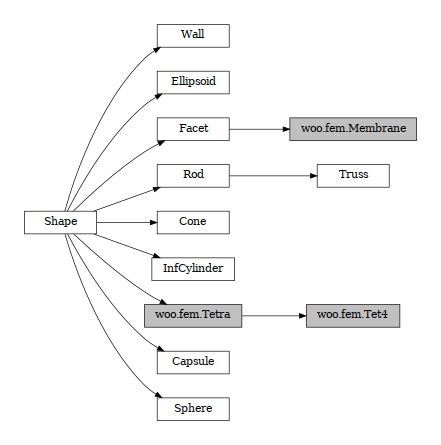class woo.dem.Shape(*args, **kwargs)

Particle geometry

1. __init__(self: woo.dem.Shape) -> None

2. __init__(self: woo.dem.Shape, *args, **kwargs) -> None

bound(= None)

Bound of the particle, for use by collision detection only

[type: shared_ptr<Bound>]

nodes(= NodeList[])

Nodes associated with this particle

[type: vector<shared_ptr<Node> >]

color(= 0.9161950680037007)

Normalized color for rendering; negative values render with wire (rather than solid), $$|\text{color}|$$>2 means invisible. (use wire, hi and visible to manipulate those)

[type: Real]

asRaw(self: woo.dem.Shape)tuple
dispHierarchy(self: woo.dem.Shape, names: bool = True)list

Return list of dispatch classes (from down upwards), starting with the class instance itself, top-level indexable at last. If names is true (default), return class names rather than numerical indices.

property dispIndex

Return class index of this instance.

property equivRadius

Volumetrically equivalent radius of this shape. Returns NaN if the shape is multinodal or infinite.

property hi

Display this shape as highlighted (blinking color); internally encoded in color.

isInside(self: woo.dem.Shape, pt: _wooEigen11.Vector3)bool

Fast predicate testing whether pt is inside or outside this shape.

lumpMassInertia(self: woo.dem.Shape, arg0: woo.core.Node, arg1: float)tuple
setFromRaw(self: woo.dem.Shape, arg0: _wooEigen11.Vector3, arg1: float, arg2: woo.core.NodeList, arg3: List[float])None
property visible

Set the shape as invisible; internally encoded in color.

property volume

Volume of this shape; returns NaN for multinodal or infinite shapes.

property wire

Display this shape as wire or non-wire (unless overrideden by GL functor); internally encoded in color.

#### InfCylinder¶

class woo.dem.InfCylinder(*args, **kwargs)

Object representing infinite plane aligned with the coordinate system (axis-aligned wall).

1. __init__(self: woo.dem.InfCylinder) -> None

2. __init__(self: woo.dem.InfCylinder, *args, **kwargs) -> None

radius(= nan)

[type: Real]

axis(= 0)

Axis of the normal; can be 0,1,2 for +x, +y, +z respectively (Node’s orientation is disregarded for walls)

[type: int]

glAB(= Vector2(nan, nan))

Endpoints between which the infinite cylinder is drawn, in local coordinate system along axis; if NaN, taken from scene view to be visible

[type: Vector2r]

static make(position, radius, axis, glAB=None, fixed=True, mass=0, color=None, wire=False, angVel=None, mat=<function defaultMaterial>, mask=5)

#### Wall¶

class woo.dem.Wall(*args, **kwargs)

Object representing infinite plane aligned with the coordinate system (axis-aligned wall).

1. __init__(self: woo.dem.Wall) -> None

2. __init__(self: woo.dem.Wall, *args, **kwargs) -> None

sense(= 0)

Which side of the wall interacts: -1 for negative only, 0 for both, +1 for positive only.

[type: int]

axis(= 0)

Axis of the normal; can be 0,1,2 for +x, +y, +z respectively (Node’s orientation is disregarded for walls)

[type: int]

glAB(= AlignedBox2((nan, nan), (nan, nan)))

Points between which the wall is drawn (if NaN, computed automatically to cover the visible part of the scene)

[type: AlignedBox2r]

static make(position, axis, sense=0, glAB=None, fixed=True, mass=0, color=None, mat=<function defaultMaterial>, visible=True, mask=3)

Parameters
• position (float-or-Vector3-or-Node) – center of the wall. If float, it is the position along given axis, the other 2 components being zero

• axis (∈{0,1,2}) – orientation of the wall normal (0,1,2) for x,y,z (sc. planes yz, xz, xy)

• sense (∈{-1,0,1}) – sense in which to interact (0: both, -1: negative, +1: positive; see woo.dem.Wall)

See woo.utils.sphere’s documentation for meaning of other parameters.

static makeBox(box, which=(1, 1, 1, 1, 1, 1), **kw)

Return box delimited by walls, created by woo.dem.Wall.make, which receives most arguments. Wall.glAB are computed automatically so that walls visually end at the edges.

Note

Since walls are infinite, they will still interact with other particle beyond this box; use woo.triangulated.box for true box with arbitrary orientation.

Parameters
• box – axis-aligned box determining positions of walls.

• which – determines which of the 6 walls are created (boolean values), in the order -x, -y, -z, +x, +y, +z. For instance, to create a box which does not have the top, say which=(1,1,1,1,1,0)).

Returns

list of Particle objects.

#### Sphere¶

class woo.dem.Sphere(*args, **kwargs)

Spherical particle.

1. __init__(self: woo.dem.Sphere) -> None

2. __init__(self: woo.dem.Sphere, *args, **kwargs) -> None

radius(= nan)

[type: Real, unit: m]

static make(center, radius, mat=<function defaultMaterial>, fixed=False, wire=False, color=None, highlight=False, mask=5, vel=None)

Create sphere with given parameters; mass and inertia computed automatically.

Parameters
Returns

Particle instance with desired characteristics.

Instance of material can be given:

>>> from woo import utils
>>> s1=utils.sphere((0,0,0),1,wire=False,color=.7,mat=ElastMat(young=30e9,density=2e3))
>>> s1.shape.wire
False
>>> s1.shape.color
0.7
>>> s1.mat.density
2000.0


Finally, material can be a callable object (taking no arguments), which returns a Material instance. Use this if you don’t call this function directly (for instance, through woo.pack.randomDensePack), passing only 1 material parameter, but you don’t want material to be shared.

For instance, randomized material properties can be created like this:

>>> import random
>>> def matFactory(): return ElastMat(young=1e10*random.random(),density=1e3+1e3*random.random())
...
>>> s2=utils.sphere([0,2,0],1,mat=matFactory)
>>> s3=utils.sphere([1,2,0],1,mat=matFactory)


#### Ellipsoid¶

class woo.dem.Ellipsoid(*args, **kwargs)

Ellipsoidal particle.

1. __init__(self: woo.dem.Ellipsoid) -> None

2. __init__(self: woo.dem.Ellipsoid, *args, **kwargs) -> None

semiAxes(= Vector3(nan, nan, nan))

Semi-principal axes.

[type: Vector3r, unit: m]

static make(center, semiAxes, ori=Quaternion((1, 0, 0), 0), angVel=None, color=None, mat=<function defaultMaterial>, fixed=False, wire=False, visible=True, mask=5, **kw)

Return an woo.dem.Ellipsoid particle.

#### Rod¶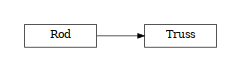class woo.dem.Rod(*args, **kwargs)

Line element without internal forces, with circular cross-section and hemi-spherical caps at both ends. Geometrically the same Capsule, but with 2 nodes.

1. __init__(self: woo.dem.Rod) -> None

2. __init__(self: woo.dem.Rod, *args, **kwargs) -> None

radius(= nan)

[type: Real]

static make(vertices, radius, fixed=True, wire=True, color=None, mat=<function defaultMaterial>, visible=True, mask=3, __class=<class 'woo.dem.Rod'>)

Create Rod with given parameters:

Parameters
• vertices – endpoints given as coordinates (Vector3) or nodes

• wire – render as wire by default

• color – color as scalar (0…1); if not given, random color is assigned

• mat – material; if not given, default material is assigned

• visible

##### Truss¶
class woo.dem.Truss(*args, **kwargs)

Describes line element (cylinder) with optional caps and with free or constrained rotations at either end.

1. __init__(self: woo.dem.Truss) -> None

2. __init__(self: woo.dem.Truss, *args, **kwargs) -> None

l0(= nan)

Initial (usually equilibrium) length

[type: Real]

axialStress(= 0.0)

Current normal stress (informative only)

[type: Real]

preStress(= 0.0)

Pre-stress (stress at zero strain)

[type: Real]

#### Facet¶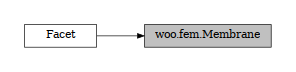class woo.dem.Facet(*args, **kwargs)

Facet (triangle in 3d) particle.

1. __init__(self: woo.dem.Facet) -> None

2. __init__(self: woo.dem.Facet, *args, **kwargs) -> None

fakeVel(= Vector3(0, 0, 0))

Fake velocity when computing contact, in global coordinates (for modeling moving surface modeled using static triangulation); only in-plane velocity is meaningful, but this is not enforced.

Note

If the x-component is NaN, the meaning is special: fakeVel is taken as zero vector and, in addition, local in-plane facet’s linear velocity at the contact is taken as zero (rather than linearly interpolated between velocity of nodes).

[type: Vector3r]

halfThick(= 0.0)

Geometric thickness (added in all directions)

[type: Real]

n21lim(= Vector3(nan, nan, nan))

Edge & vertex contact: limit value for dot-product with normal (dot-product of normal with in-plane angle of neighboring facet, perpendicular to the edge).

[type: Vector3r]

area(self: woo.dem.Facet)float

Return surface area of the facet

computeNeighborAngles(self: woo.dem.Facet)None

Compute n21Min using beighboring facets so that contact direction can be adjusted (only some Facet-X functors support that currently).

getCentroid(self: woo.dem.Facet)_wooEigen11.Vector3

Return centroid of the facet

getNormal(self: woo.dem.Facet)_wooEigen11.Vector3

Return normal vector of the facet

static make(vertices, fakeVel=None, halfThick=0.0, fixed=True, wire=True, color=None, highlight=False, mat=<function defaultMaterial>, visible=True, mask=3, flex=None, __class=<class 'woo.dem.Facet'>)

Create facet with given parameters.

Parameters
• vertices ([Vector3,Vector3,Vector3]) – coordinates of vertices in the global coordinate system.

• wire (bool) – if True, facets are shown as skeleton; otherwise facets are filled

See woo.utils.sphere’s documentation for meaning of other parameters.

outerEdgeNormals(self: woo.dem.Facet)List[_wooEigen11.Vector3]

Return outer edge normal vectors

#### Cone¶

class woo.dem.Cone(*args, **kwargs)

Line element without internal forces, with circular cross-section and hemi-spherical caps at both ends. Geometrically the same Capsule, but with 2 nodes.

1. __init__(self: woo.dem.Cone) -> None

2. __init__(self: woo.dem.Cone, *args, **kwargs) -> None

radii(= Vector2(nan, nan))

Radii of the cone. One of them can be zero (for full cone). When both radii are non-zero, the result is conical frustum.

[type: Vector2r]

static make(vertices, radii, *, fixed=True, wire=False, color=None, angVel=None, mat=<function defaultMaterial>, visible=True, mask=3)

Create cone with given parameters.

#### Capsule¶

class woo.dem.Capsule(*args, **kwargs)

Cylinder with half-spherical caps on both sides, Mindowski sum of segment with sphere.

1. __init__(self: woo.dem.Capsule) -> None

2. __init__(self: woo.dem.Capsule, *args, **kwargs) -> None

radius(= nan)

Radius of the capsule – of half-spherical caps and also of the middle part.

[type: Real, unit: m]

shaft(= nan)

Length of the middle segment

[type: Real, unit: m]

endPt(self: woo.dem.Capsule, i: int)_wooEigen11.Vector3

Return one of capsule endpoints. The first (negative on local $$x$$-axis) is returned with *i=0, otherwise the second one is returned.

static make(center, radius, shaft, ori=Quaternion((1, 0, 0), 0), fixed=False, color=None, wire=False, mat=<function defaultMaterial>, mask=5)

### Material properties¶

TODO

#### Material¶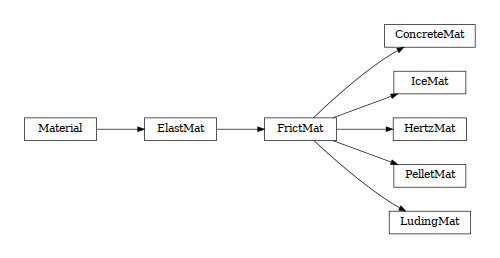class woo.dem.Material(*args, **kwargs)

Particle material

1. __init__(self: woo.dem.Material) -> None

2. __init__(self: woo.dem.Material, *args, **kwargs) -> None

density(= 1000.0)

Density

[type: Real, unit: kg/m³]

id(= -1)

Some number identifying this material; used with MatchMaker objects, useless otherwise

[type: int, not shown in the UI]

dispHierarchy(self: woo.dem.Material, names: bool = True)list

Return list of dispatch classes (from down upwards), starting with the class instance itself, top-level indexable at last. If names is true (default), return class names rather than numerical indices.

property dispIndex

Return class index of this instance.

#### ElastMat¶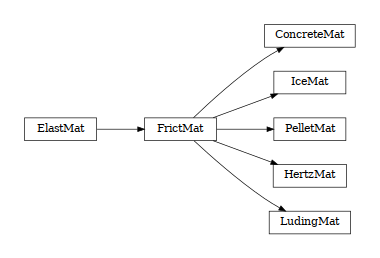class woo.dem.ElastMat(*args, **kwargs)

Elastic material with contact friction. See also ElastMat.

1. __init__(self: woo.dem.ElastMat) -> None

2. __init__(self: woo.dem.ElastMat, *args, **kwargs) -> None

young(= 1000000000.0)

Young’s modulus

[type: Real, unit: Pa]

##### FrictMat¶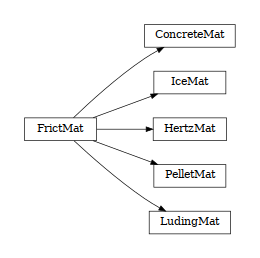class woo.dem.FrictMat(*args, **kwargs)

Elastic material with contact friction. See also ElastMat.

1. __init__(self: woo.dem.FrictMat) -> None

2. __init__(self: woo.dem.FrictMat, *args, **kwargs) -> None

tanPhi(= 0.5)

Tangent of internal friction angle.

[type: Real]

ktDivKn(= 0.2)

Ratio of tangent and shear modulus on contact.

[type: Real]

###### PelletMat¶
class woo.dem.PelletMat(*args, **kwargs)

Material describing pellet behavior; see Law2_L6Geom_PelletPhys_Pellet for details of the material model.

1. __init__(self: woo.dem.PelletMat) -> None

2. __init__(self: woo.dem.PelletMat, *args, **kwargs) -> None

normPlastCoeff(= 0.0)

Coefficient $$\alpha$$ in the normal yield function; non-positive deactivates.

[type: Real]

kaDivKn(= 0.0)

Ratio of $$\frac{k_A}{K_N}$$ for the adhesion function; non-positive deactivates.

[type: Real]

PelletAgglomerator
class woo.dem.PelletAgglomerator(*args, **kwargs)

Compute agglomeration of pellets due to contact some special particles, or wearing due to impacts (only applies to particles with PelletMat.

1. __init__(self: woo.dem.PelletAgglomerator) -> None

2. __init__(self: woo.dem.PelletAgglomerator, *args, **kwargs) -> None

agglomSrcs(= _8ParticleList[])

Sources of agglomerating mass; particles in contact with this source will have their radius increased based on their relative angular velocity.

[type: vector<shared_ptr<Particle>>]

massIncPerRad(= nan)

Increase of sphere mass per one radian of rolling (radius is increased in such way that mass increase is satisfied).

[type: Real]

dampHalfLife(= -10000.0)

Half-life for rotation damping (includes both rolling and twist); if negative, relative to the (initial) woo.core.Scene.dt; zero deactivates damping. Half-life is $$t_{1/.2}=\frac{\ln 2}{\lambda}$$ where $$\lambda$$ is decay coefficient applied as $$\d\omega=-\lambda\omega$$ (see http://en.wikipedia.org/wiki/Exponential_decay for details).

[type: Real]

currRate(= 0.0)

Current rate of mass increase due to agglomeration.

[type: Real]

currRateSmooth(= 0.001)

Smoothing coefficient for currRate ∈〈0,1〉 (should be rather low, since this engine runs at every step).

[type: Real]

mass(= 0.0)

Total cumulative mass increase due to agglomeration.

[type: Real]

###### LudingMat¶
class woo.dem.LudingMat(*args, **kwargs)

Material for luding-contact-model.

1. __init__(self: woo.dem.LudingMat) -> None

2. __init__(self: woo.dem.LudingMat, *args, **kwargs) -> None

► Normal

k1DivKn(= 0.5)

[type: Real]

kaDivKn(= 0.2)

[type: Real]

deltaLimRel(= 0.1)

Maximum plasticity depth relative to minimum radius of contacting particles.

[type: Real]

viscN`(= 0.0)

Normal viscous coefficient.

[type: Real]

► Tangential